# Axis in Xamarin.iOS Chart(SfChart)

1 Mar 202224 minutes to read

Charts typically have two axes that are used to measure and categorize data: a vertical (Y) axis, and a horizontal (X) axis.

Vertical(Y) axis uses numeric or logarithmic scale. Horizontal(X) axis supports the following types of scale:

• Category
• Numeric
• Date time
• Logarithmic Axis

The following APIs are available in ChartAxis:

## Category Axis

Category axis displays text labels instead of numbers.

• C#
• ``````SFCategoryAxis xAxis = new SFCategoryAxis ();

chart.PrimaryAxis 	 = xAxis;``````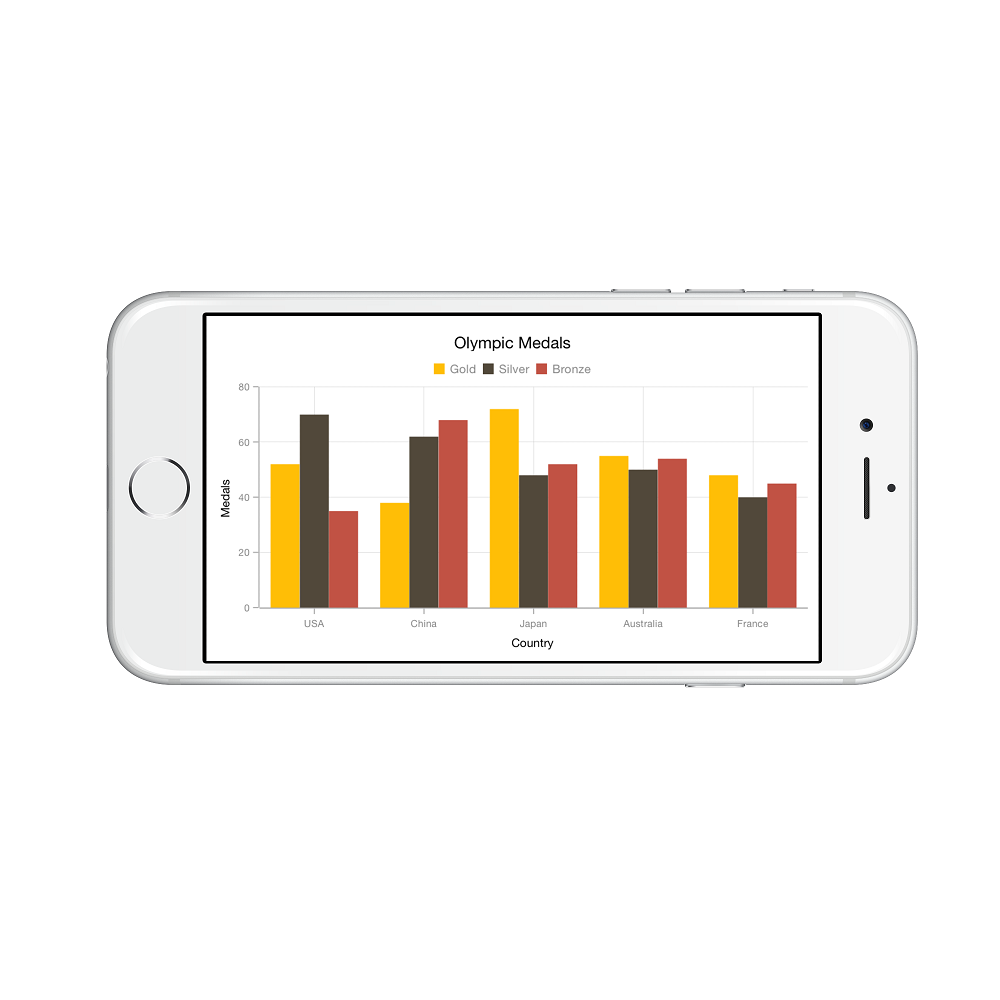### Placing labels between ticks

Labels in category axis can be placed between the ticks by setting `LabelPlacement` to `SFChartLabelPlacement.BetweenTicks`. Default value of `LabelPlacement` property is `SFChartLabelPlacement.OnTicks` i.e. labels will be placed on the ticks by default.

• C#
• ``xAxis.LabelPlacement = SFChartLabelPlacement.BetweenTicks;``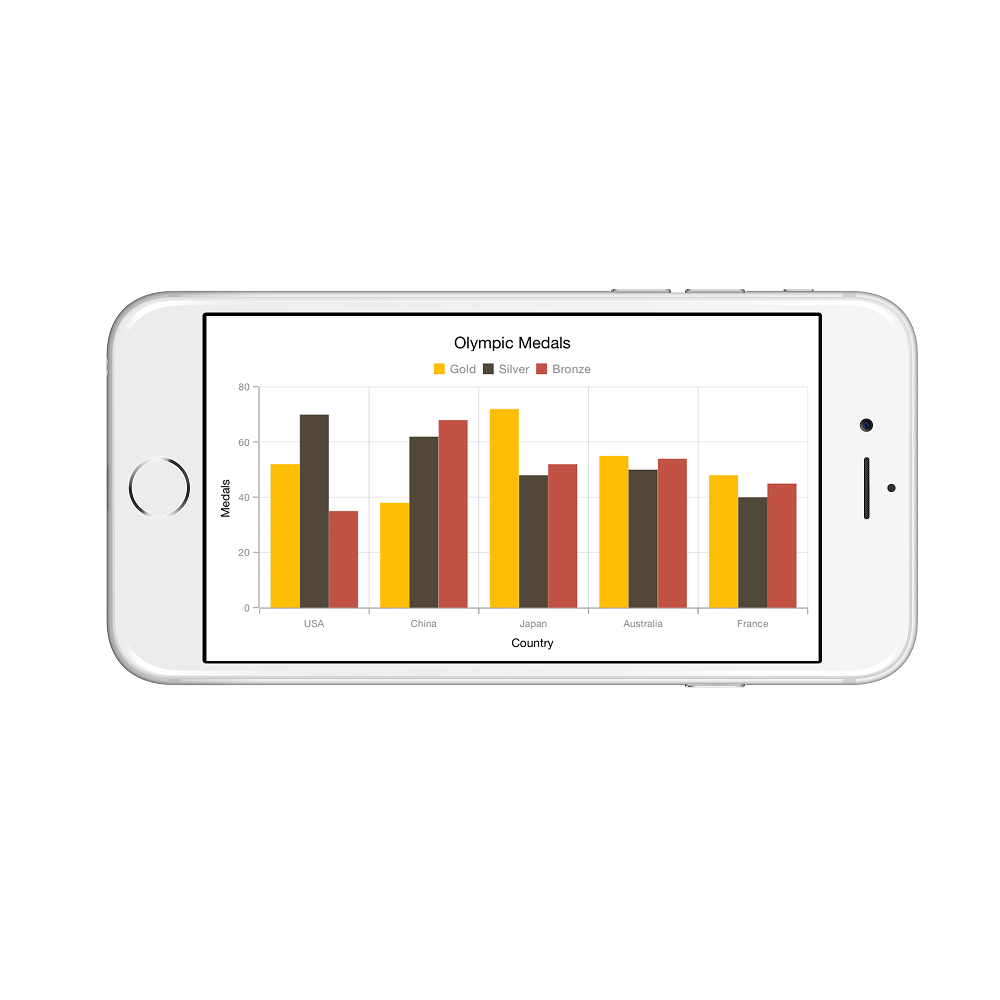### Displaying labels after a fixed interval

To display labels after a fixed interval n, you can set `Interval` property of `SFAxis` as n. Default value of interval is 1 i.e. all the labels will be displayed by default.

• C#
• ``xAxis.Interval = new NSNumber (2);``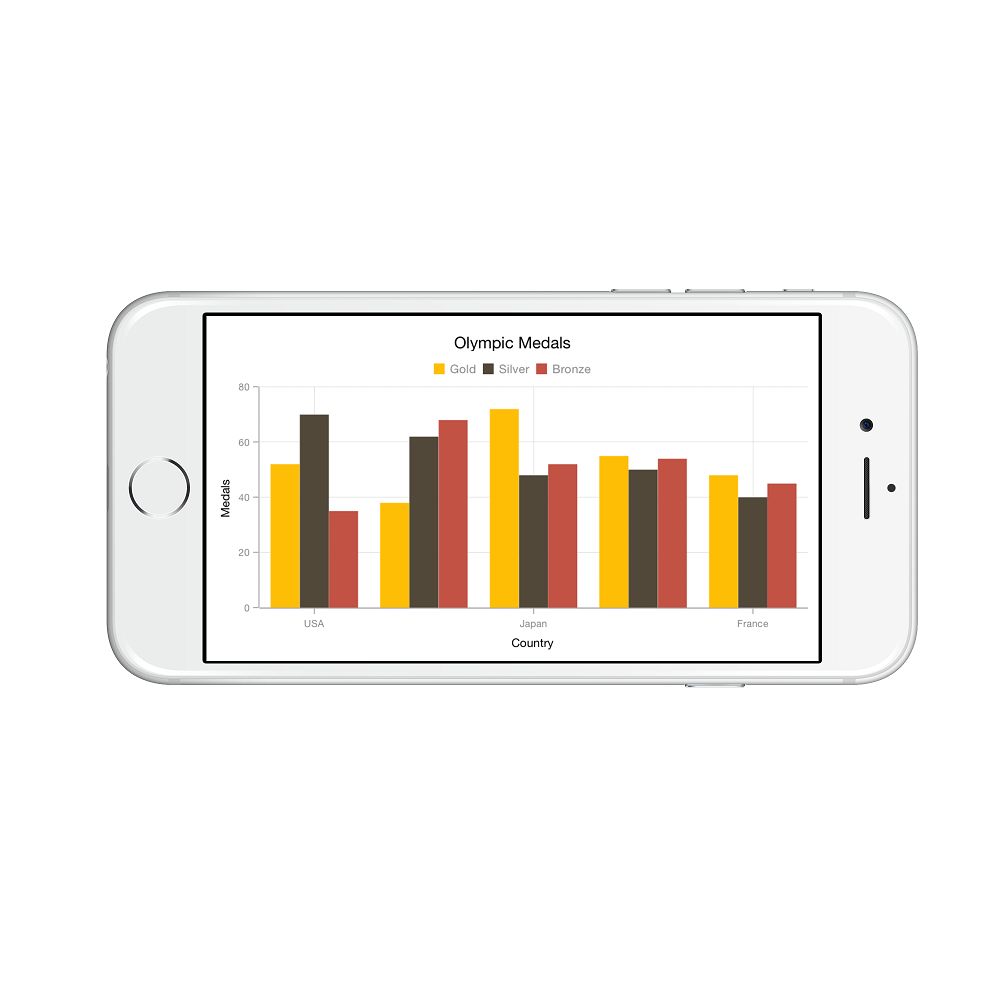### Indexed category axis

Category axis can also be rendered based on the index values of data source by setting the `ArrangeByIndex` property to true in the axis.

• C#
• ``````[C#]

chart.PrimaryAxis = new SFCategoryAxis()
{
ArrangeByIndex = false
};

SFColumnSeries series1 = new SFColumnSeries()
{
ItemsSource = view.Data1,
XBindingPath = "Country",
YBindingPath = "Year2016"
};
SFColumnSeries series2 = new SFColumnSeries()
{
ItemsSource = view.Data2,
XBindingPath = "Country",
YBindingPath = "Year2016",
};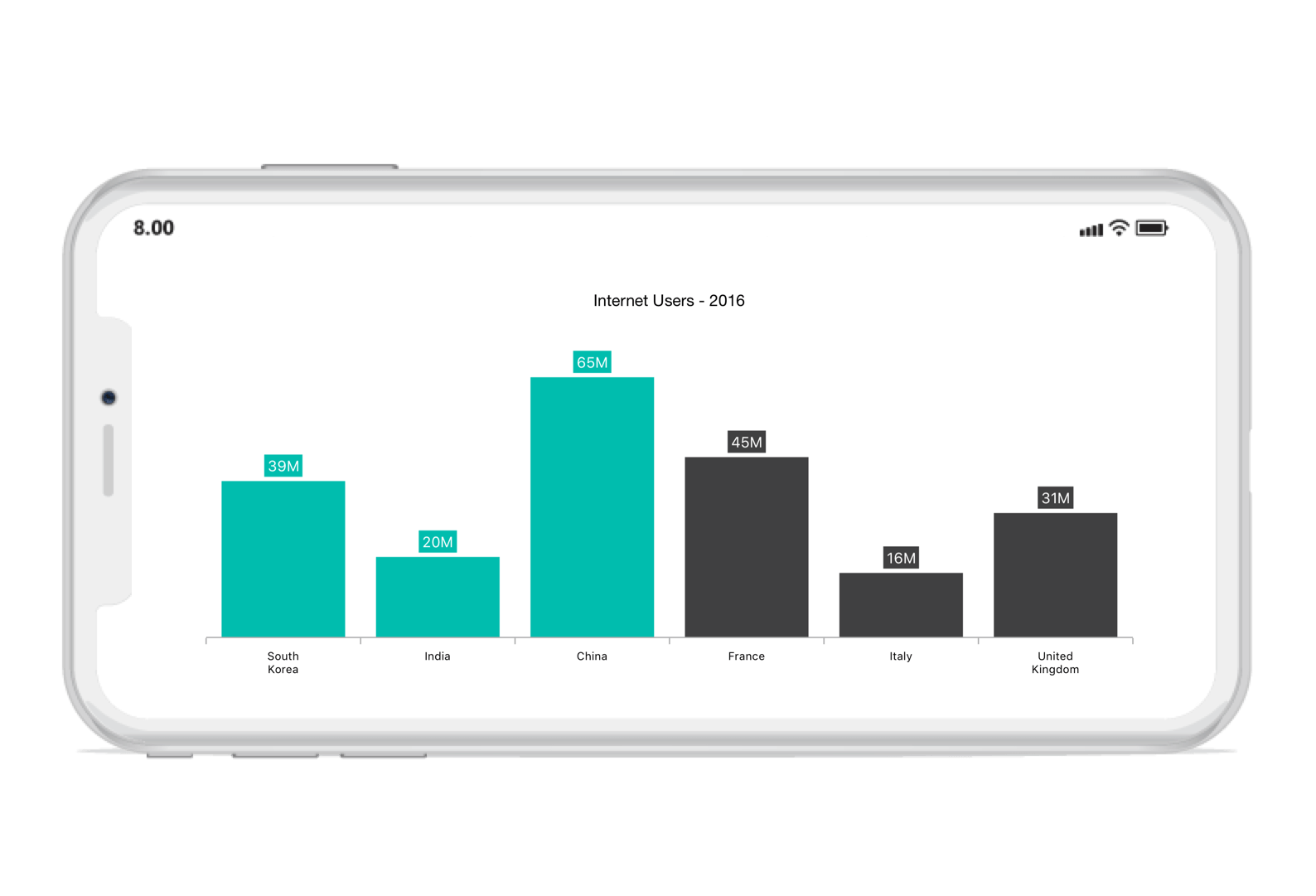## Numeric Axis

Numeric axis uses numerical scale and displays numbers as labels.

• C#
• ``````SFNumericalAxis xAxis = new SFNumericalAxis ();

chart.PrimaryAxis     = xAxis;``````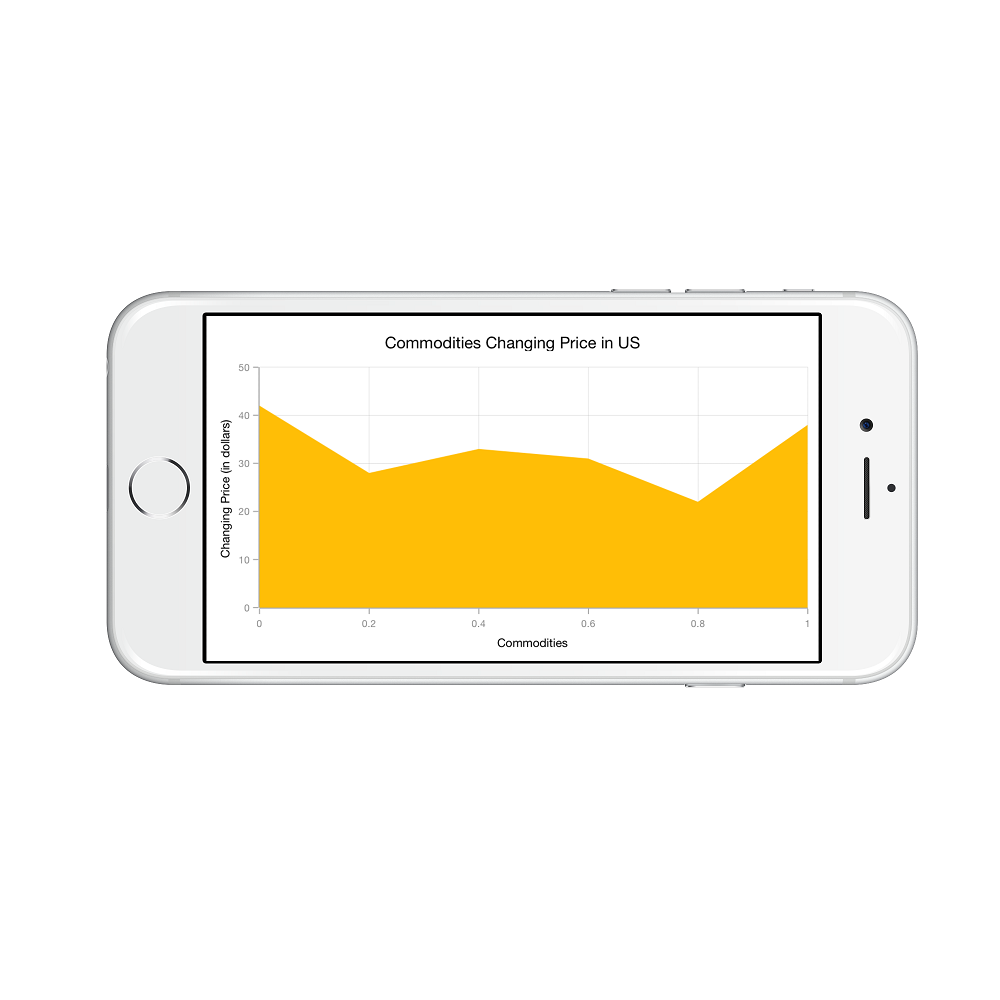### Customizing numeric range

To customize the range of an axis, you can use the `Minimum` and `Maximum` properties of `SFNumericalAxis`. By default, range will be calculated automatically based on the provided data.

• C#
• ``````SFNumericalAxis yAxis 	= new SFNumericalAxis ();

chart.SecondaryAxis     = yAxis;

yAxis.Minimum           = new NSNumber (10);

yAxis.Maximum           = new NSNumber (50);``````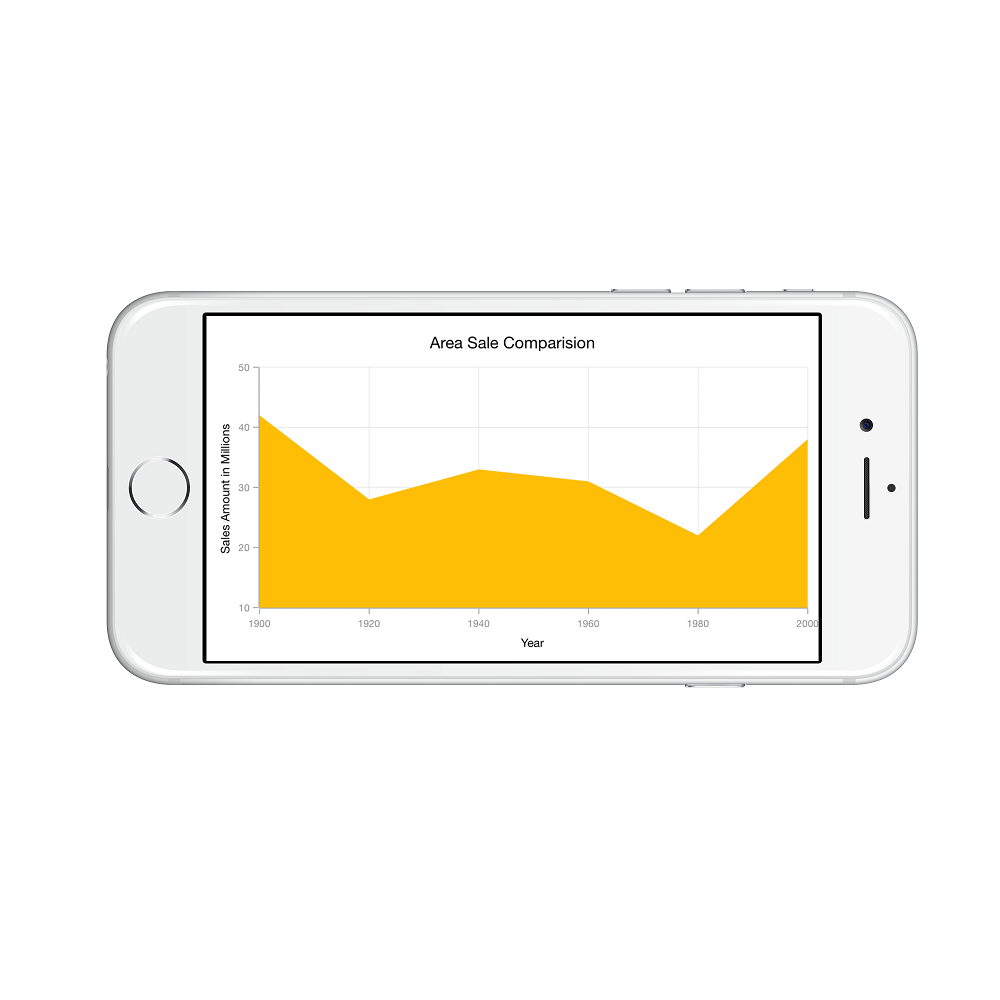### Customizing numeric interval

Axis interval can be customized using the `Interval` property of `SFAxis`. By default, interval will be calculated based on the minimum and maximum value of the provided data.

• C#
• ``yAxis.Interval = new NSNumber (5);``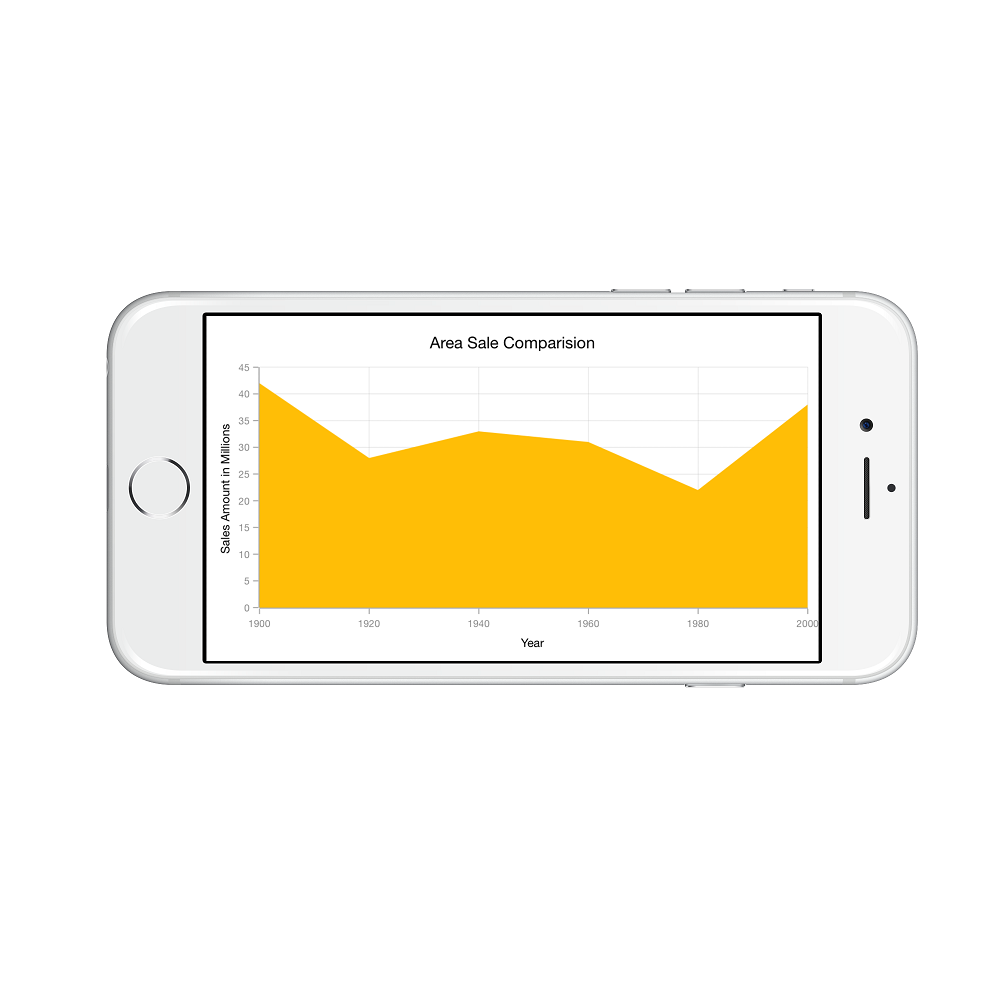### Apply padding to the range

Padding can be applied to the minimum and maximum extremes of the axis range by using `RangePadding` property. Numeric axis supports the following types of padding.

• None
• Round
• Normal
• RoundStart
• RoundEnd
• PrependInterval
• AppendInterval

None

When the value of `RangePadding` property is `SFChartNumericalPadding.None`, padding will not be applied to the axis. This is also the default value of `RangePadding` for horizontal axis.

• C#
• ``yAxis.RangePadding = SFChartNumericalPadding.None;``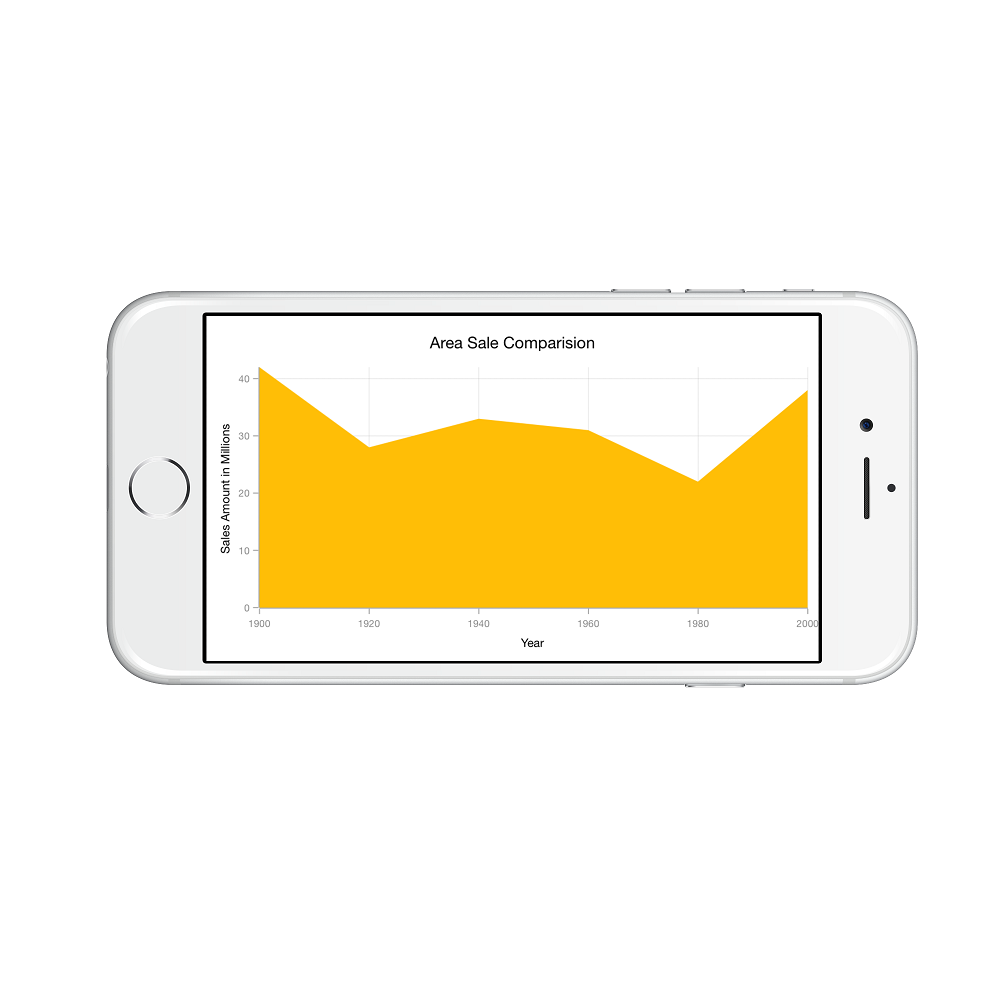Round

When the value of `RangePadding` property is `SFChartNumericalPadding.Round`, axis range will be rounded to the nearest possible value by the interval.

• C#
• ``yAxis.RangePadding = SFChartNumericalPadding.Round;``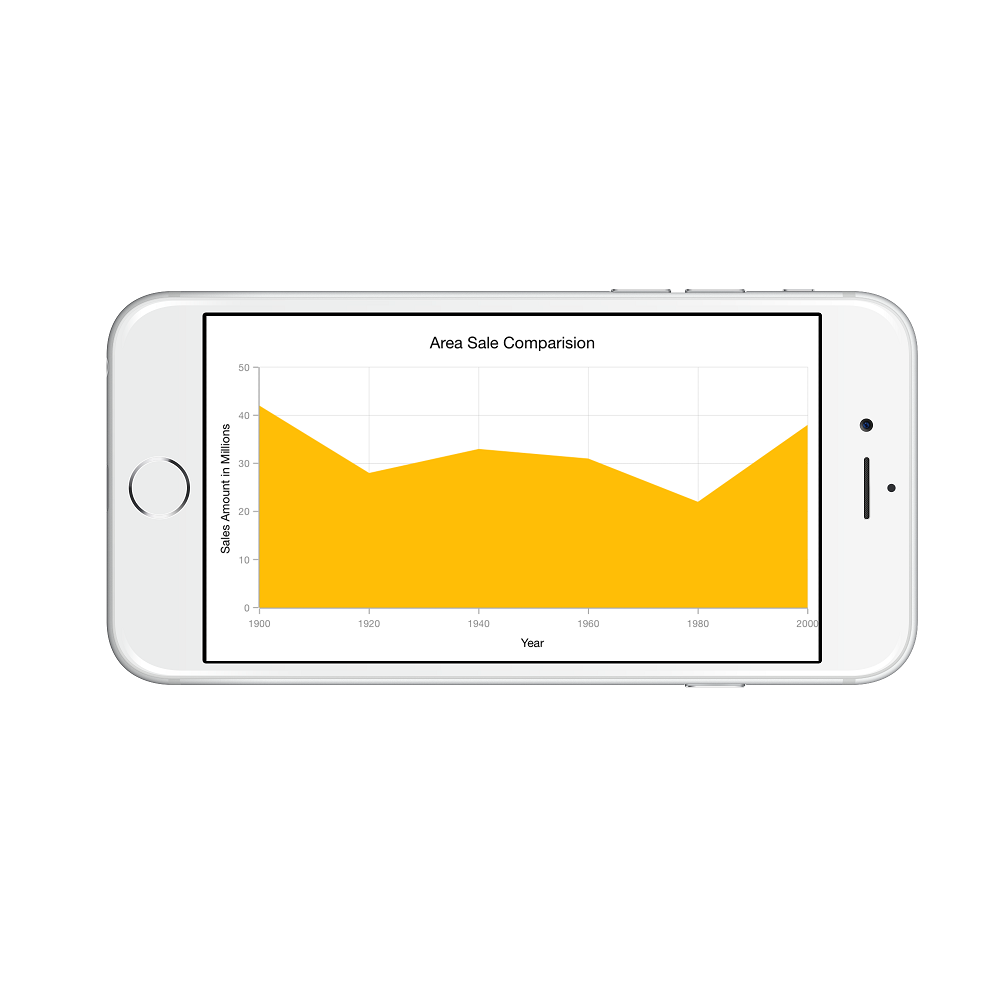When the value of `RangePadding` property is `SFChartNumericalPadding.Additional`, axis range will be rounded and an interval of the axis will be added as padding to the minimum and maximum values of the range.

• C#
• ``yAxis.RangePadding = SFChartNumericalPadding.Additional;``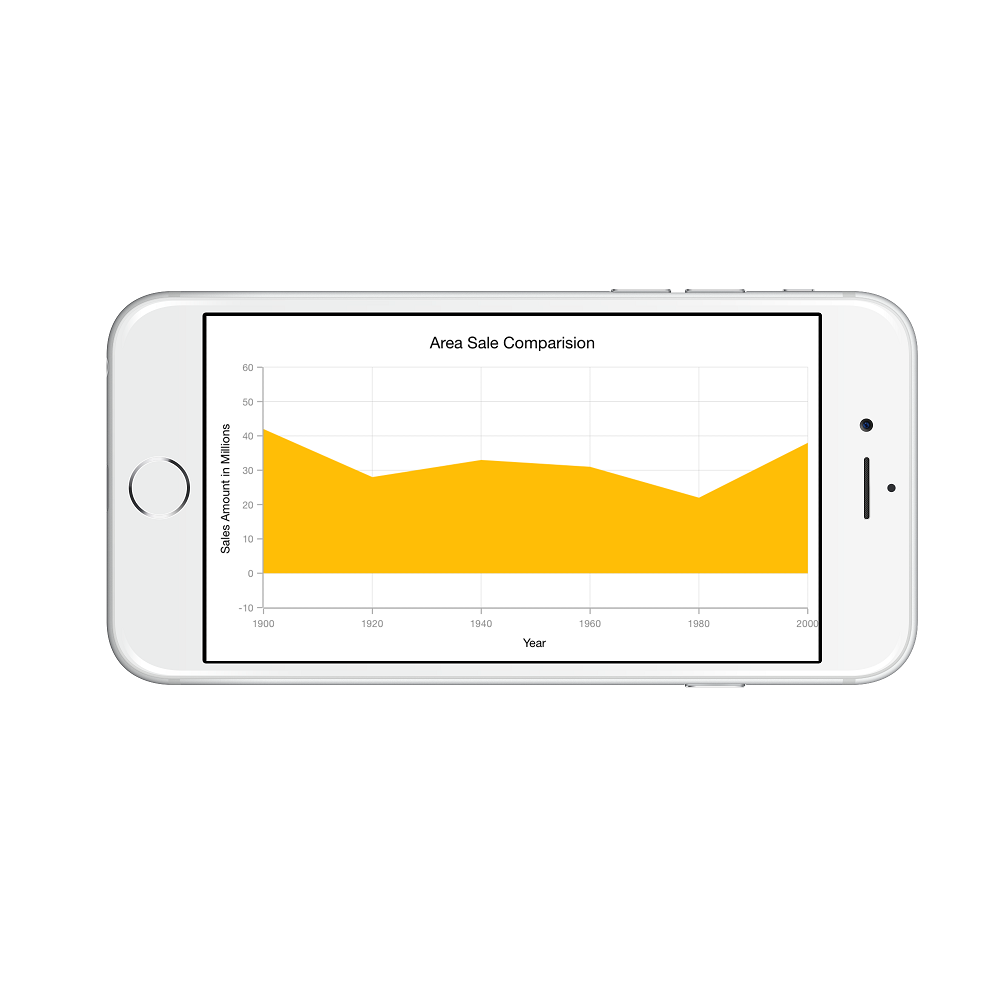Normal

When the value of `RangePadding` property is `SFChartNumericalPadding.Normal`, range will be calculated for the axis based on the best readability of the data. This is also the default for vertical axis.

• C#
• ``yAxis.RangePadding = SFChartNumericalPadding.Normal;``RoundStart

When the value of `RangePadding` property is `SFChartNumericalPadding.RoundStart`, axis range will be rounded in the start to the nearest possible value by the interval.

• C#
• ``yAxis.RangePadding = SFChartNumericalPadding.RoundStart;``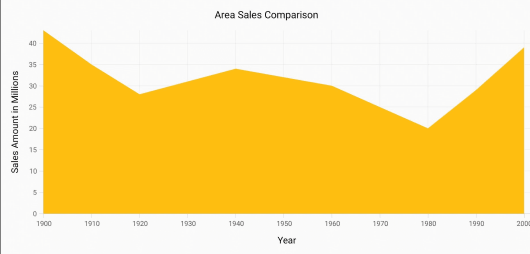RoundEnd

When the value of `RangePadding` property is `SFChartNumericalPadding.RoundEnd`, axis range will be rounded in the end to the nearest possible value by the interval.

• C#
• ``yAxis.RangePadding = SFChartNumericalPadding.RoundEnd;``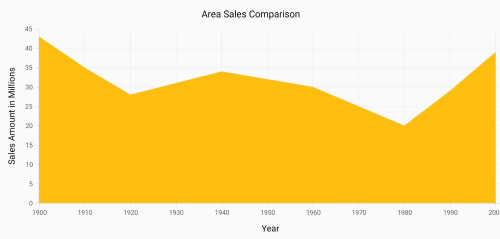PrependInterval

When the value of `RangePadding` property is `SFChartNumericalPadding.PrependInterval`, axis range will be rounded and an interval of the axis will be added in the start as padding to the minimum values of the range.

• C#
• ``yAxis.RangePadding = SFChartNumericalPadding.PrependInterval;``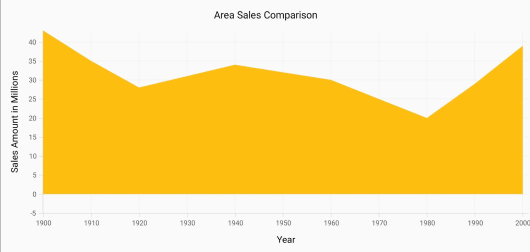AppendInterval

When the value of `RangePadding` property is `SFChartNumericalPadding.AppendInterval`, axis range will be rounded and an interval of the axis will be added in the end as padding to the maximum values of the range.

• C#
• ``yAxis.RangePadding = SFChartNumericalPadding.AppendInterval;``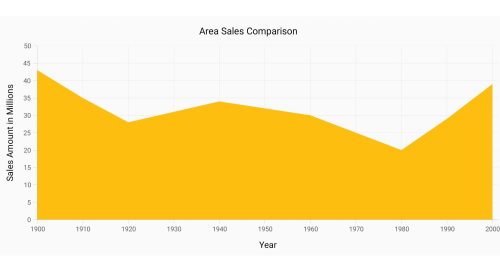## Date Time Axis

Date time axis uses date time scale and displays date time values as axis labels in specified format.

• C#
• ``````SFDateTimeAxis xAxis = new SFDateTimeAxis ();

chart.PrimaryAxis    = xAxis;``````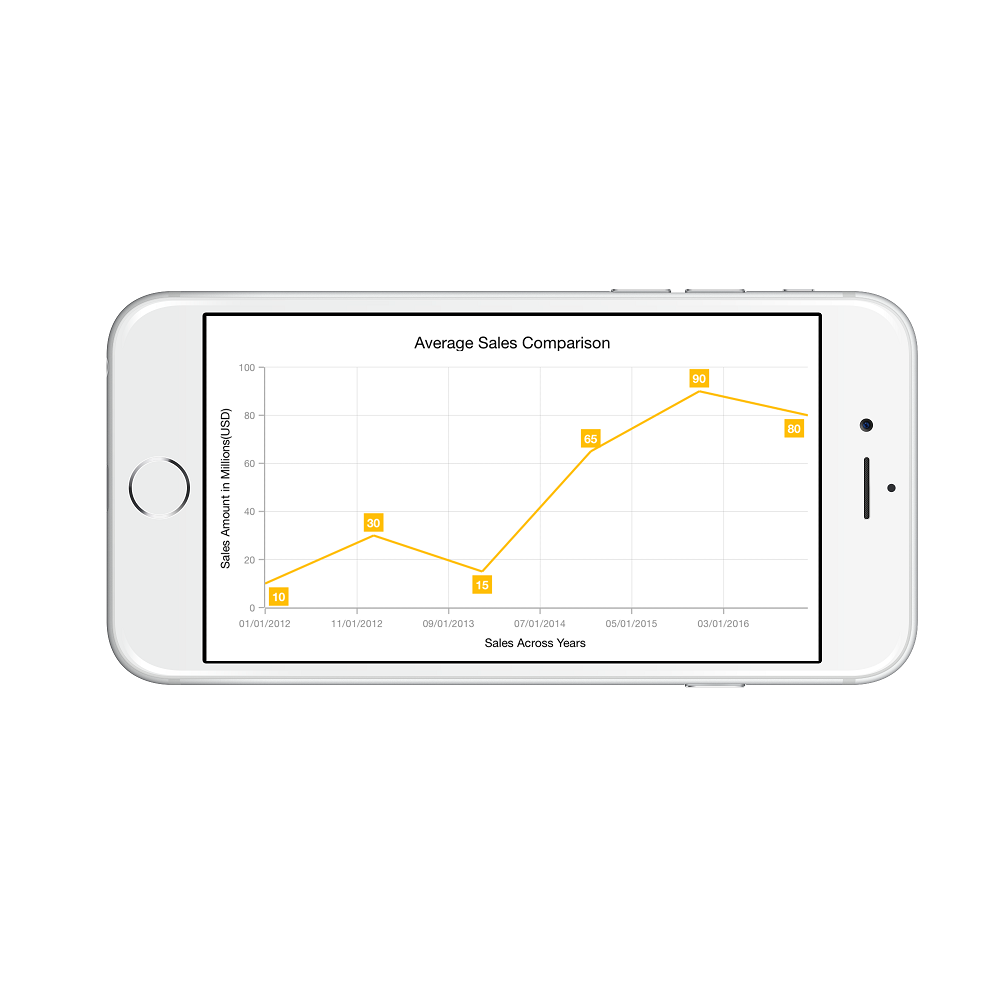### Customizing date time range

To customize the range of an axis, you can use the `Minimum` and `Maximum` properties of `SFDateTimeAxis`. By default, range will be calculated automatically based on the provided data.

• C#
• ``````SFDateTimeAxis primaryAxis = new SFDateTimeAxis();
NSCalendar calendar = new NSCalendar(NSCalendarType.Gregorian);
primaryAxis.Minimum = calendar.DateFromComponents(new NSDateComponents() {Year = 2010, Month = 1, Day = 1 });
primaryAxis.Maximum = calendar.DateFromComponents(new NSDateComponents() {Year = 2015, Month = 12, Day = 31 });``````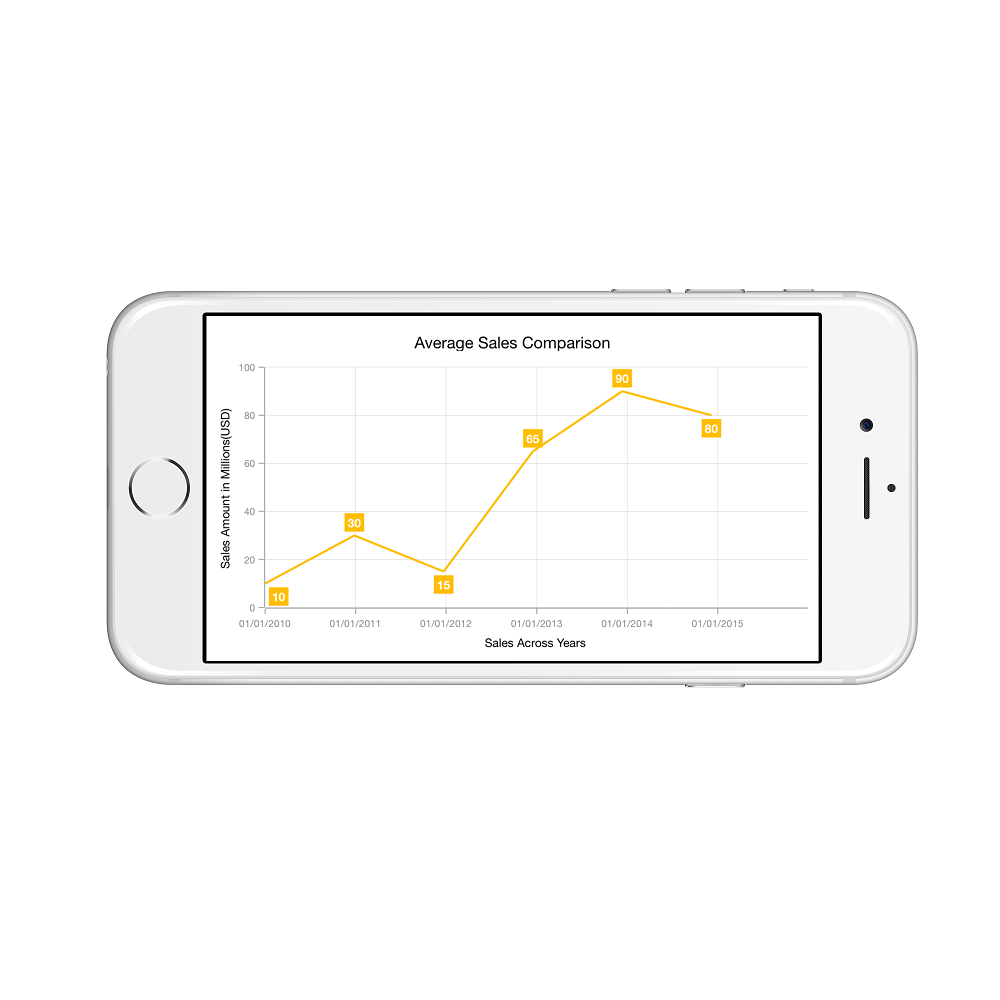### Date time intervals

Date time intervals can be customized using `Interval` and `IntervalType` properties of the `SFDateTimeAxis`. For example, setting `Interval` as 2 and `IntervalType` as `SFChartDateTimeIntervalType.Years` will consider 2 years as interval.

Essential Chart supports the following types of interval for date time axis

• Years
• Months
• Days
• Hours
• Minutes
• Seconds
• Milliseconds
• C#
• ``````xAxis.IntervalType = SFChartDateTimeIntervalType.Months;

xAxis.Interval     = new NSNumber (6);``````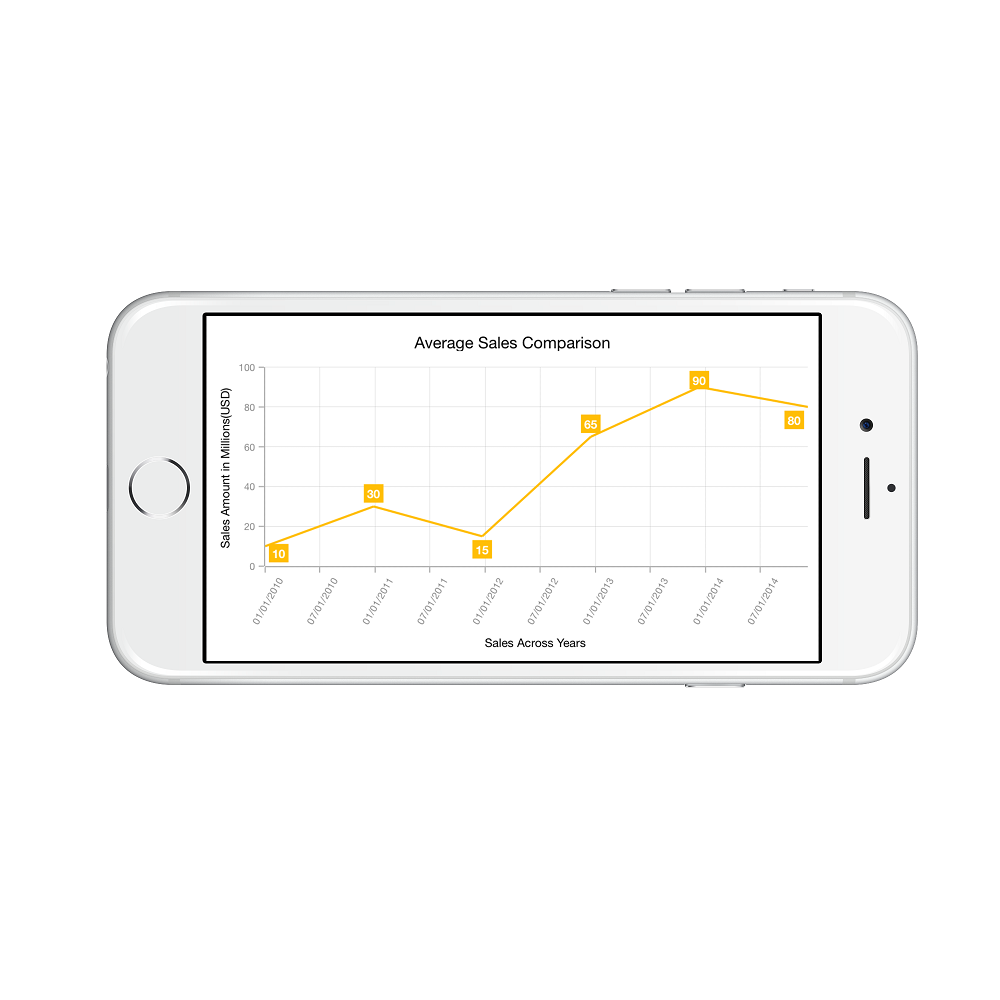### Apply padding to the range

Padding can be applied to the minimum and maximum extremes of the range by using `RangePadding` property. Date time axis supports the following types of padding:

• None
• Round
• RoundStart
• RoundEnd
• PrependInterval
• AppendInterval

None

When the value of `RangePadding` property is `SFChartDateTimePadding.None`, padding will not be applied to the axis. This is also the default value of `RangePadding`.

• C#
• ``xAxis.RangePadding = SFChartDateTimePadding.None;``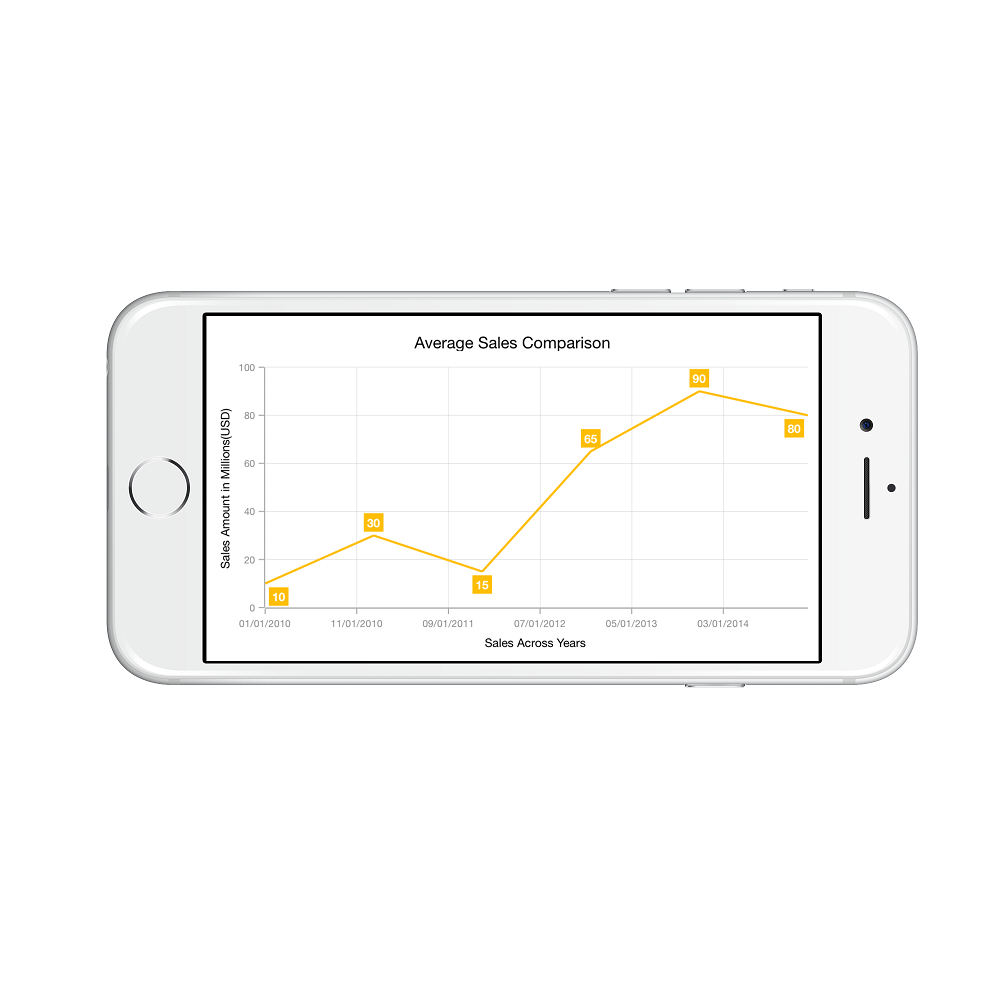Round

When the value of `RangePadding` property is `SFChartDateTimePadding.Round`, axis range will be rounded to the nearest possible date time value.

• C#
• ``xAxis.RangePadding = SFChartDateTimePadding.Round;``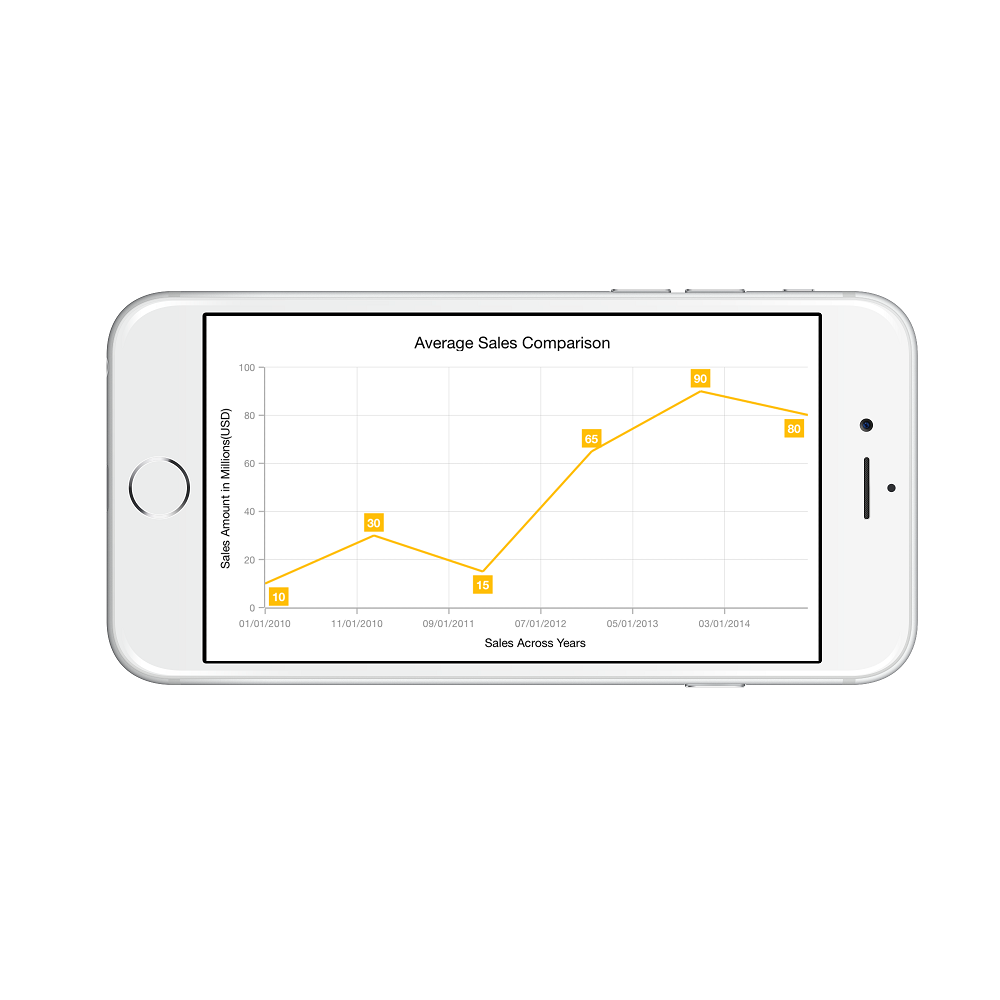When the value of `RangePadding` property is `SFChartDateTimePadding.Additional`, range will be rounded and date time interval of the axis will be added as padding to the minimum and maximum extremes of the range.

• C#
• ``xAxis.RangePadding = SFChartDateTimePadding.Additional;``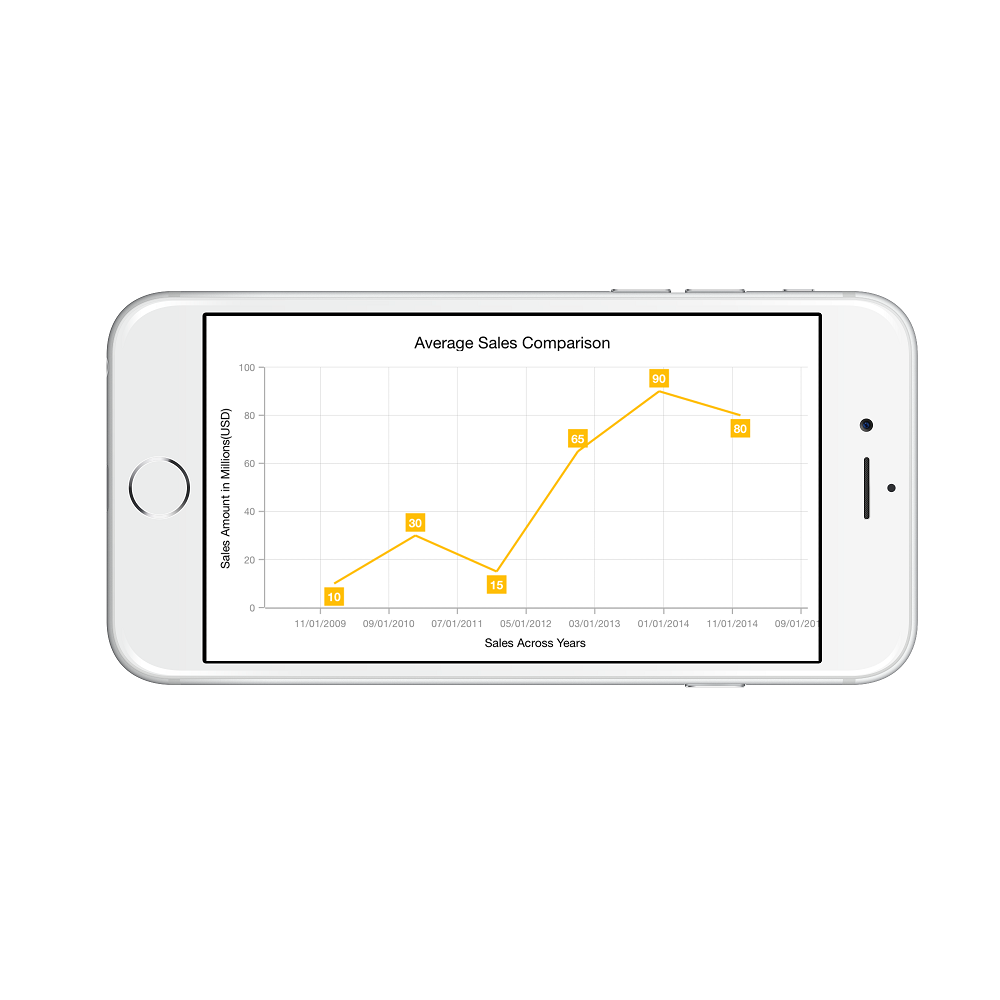RoundStart

When the value of `RangePadding` property is `SFChartDateTimePadding.RoundStart`, axis range will be rounded in the start to the nearest possible date time value.

• C#
• ``xAxis.RangePadding = SFChartDateTimePadding.RoundStart;``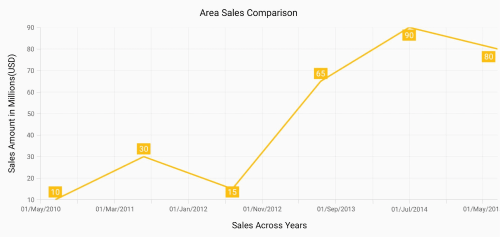RoundEnd

When the value of `RangePadding` property is `SFChartDateTimePadding.RoundEnd`, axis range will be rounded in the end to the nearest possible date time value.

• C#
• ``xAxis.RangePadding = SFChartDateTimePadding.RoundEnd;``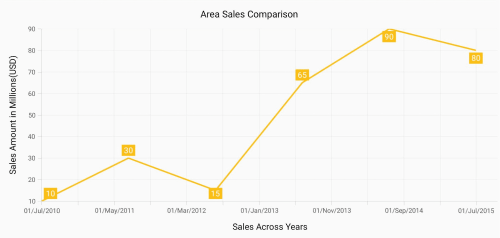PrependInterval

When the value of `RangePadding` property is `SFChartDateTimePadding.PrependInterval`, axis range will be rounded and date time interval of the axis will be added in the start as padding to the minimum extremes of the range.

• C#
• ``xAxis.RangePadding = SFChartDateTimePadding.PrependInterval;``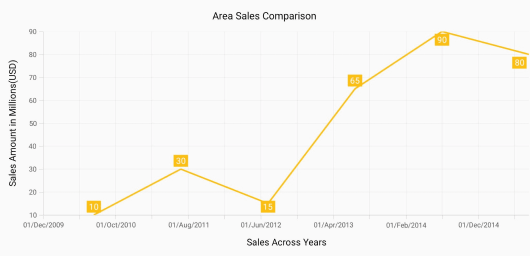AppendInterval

When the value of `RangePadding` property is `SFChartDateTimePadding.AppendInterval`, axis range will be rounded and date time interval of the axis will be added in the end as padding to the maximum extremes of the range.

• C#
• ``xAxis.RangePadding = SFChartDateTimePadding.AppendInterval;``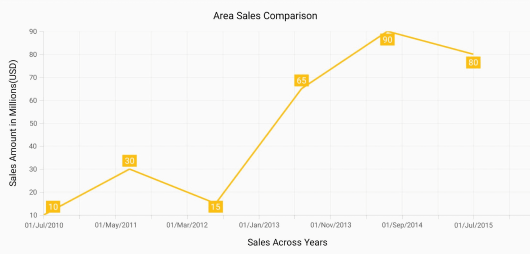## Date-time category axis

The `DateTimeCategoryAxis` is a unique type of axis used mainly with financial series. Like CategoryAxis, all the data points are plotted with equal spaces by removing space for missing dates. Intervals and ranges for the axis are calculated similar to DateTimeAxis. There will be no visual gaps between points even when the difference between two points is more than a year. The following APIs are used to customize the interval of DateTimeCategoryAxis.

`Interval` - Gets or sets the double value that represents the interval between the labels.
`IntervalType` - Gets or sets the DateTimeIntervalType that represents the type of the interval to be displayed.

• C#
• ``````SFDateTimeCategoryAxis xAxis = new SFDateTimeCategoryAxis();
xAxis.Interval = new NSNumber(1);
xAxis.IntervalType = SFChartDateTimeIntervalType.Months;
chart.PrimaryAxis = xAxis;``````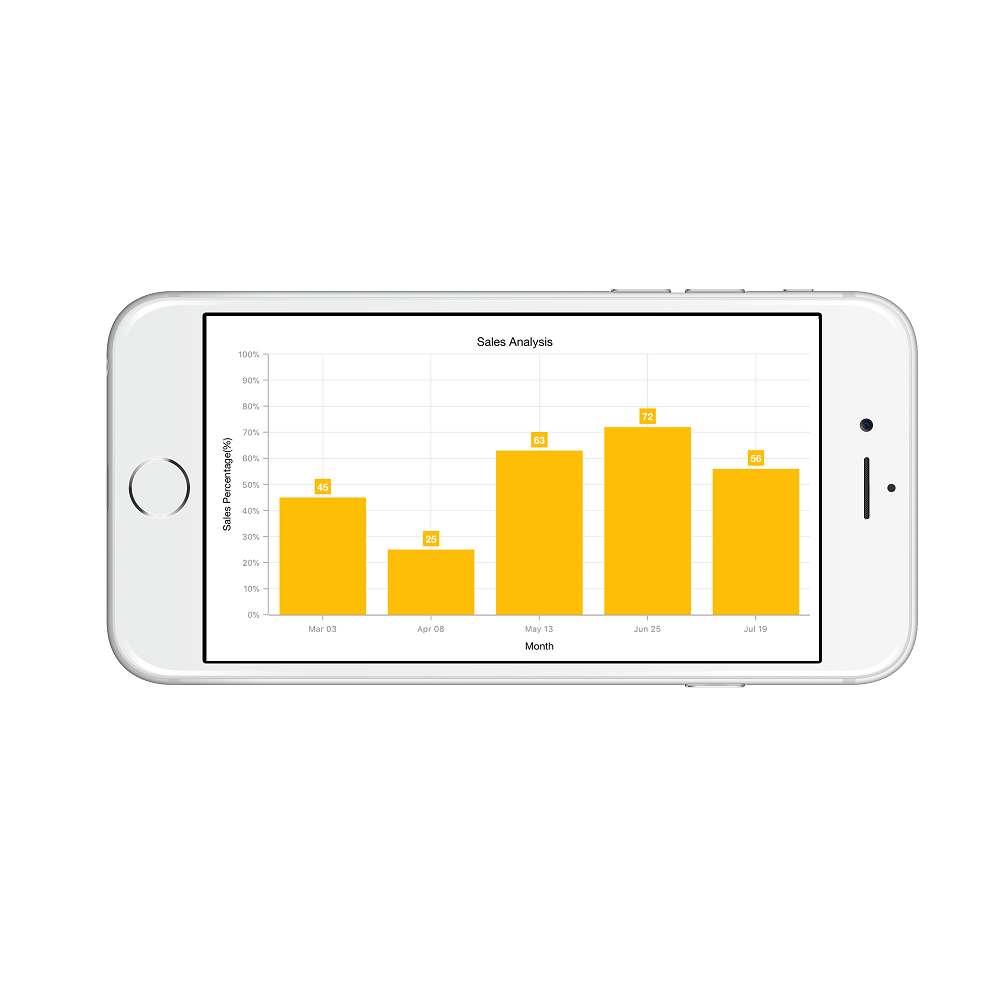## Logarithmic Axis

Logarithmic axis uses logarithmic scale and displays numbers as axis labels.

• C#
• ``````SFLogarithmicAxis yAxis    = new SFLogarithmicAxis ();
chart.SecondaryAxis        = yAxis;``````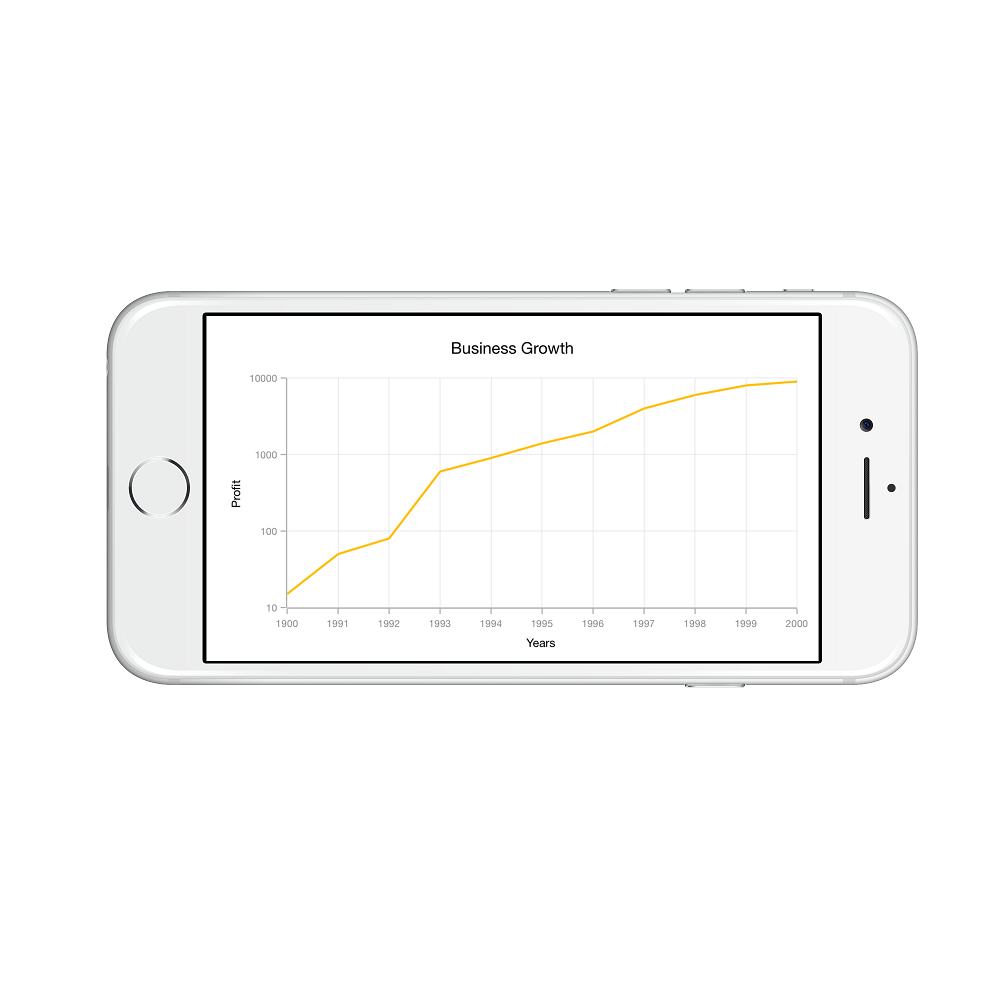### Customizing the logarithmic range

To customize the range of log axis, you can use the `Minimum` and `Maximum` properties of `LogarithmicAxis`. By default, nice range will be calculated automatically based on the provided data.

• C#
• ``````SFLogarithmicAxis yAxis         = new SFLogarithmicAxis ();
yAxis.Minimum                   = new NSNumber(100);
yAxis.Maximum                   = new NSNumber(10000);
chart.SecondaryAxis             = yAxis;``````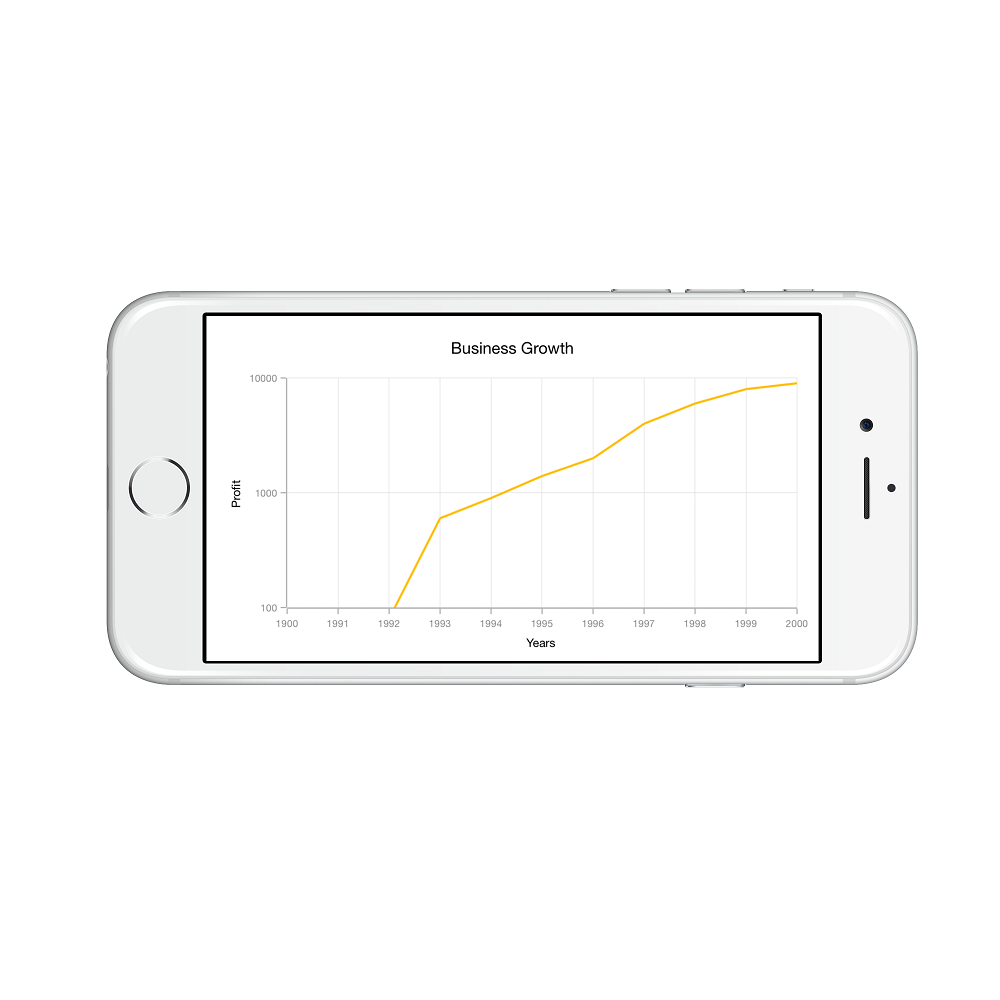### Customizing the logarithmic base

To customize the log base value, you can use `LogarithmicBase` property.

• C#
• ``````SFLogarithmicAxis yAxis          = new SFLogarithmicAxis ();
yAxis.LogarithmicBase            =  2;
chart.SecondaryAxis              = yAxis;``````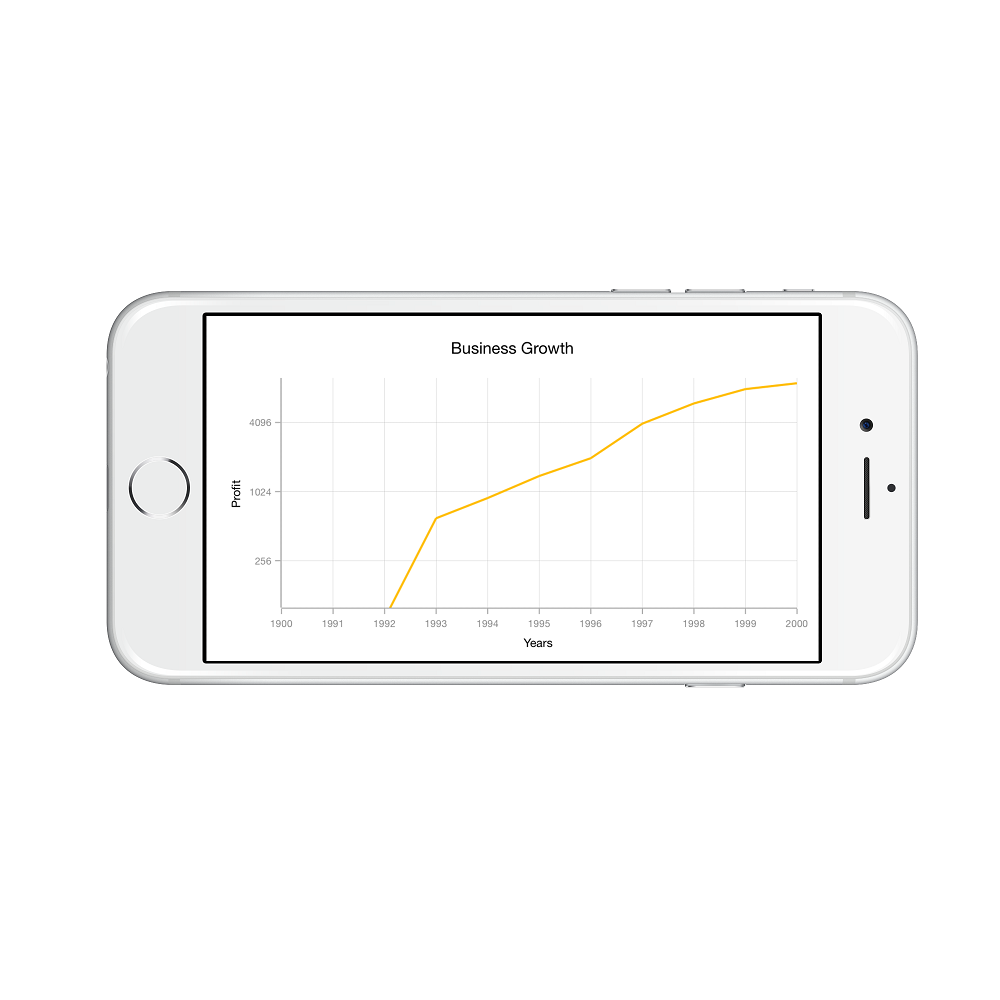## Common axis features

Customization of features such as axis title, labels, grid lines and tick lines are common to all the axes. Each of these features are explained in this section.

### Axis Visibility

Axis visibility can be controlled using the `Visible` property of axis. Default value of `Visible` property is `True`.

• C#
• ``chart.SecondaryAxis.Visible = false;``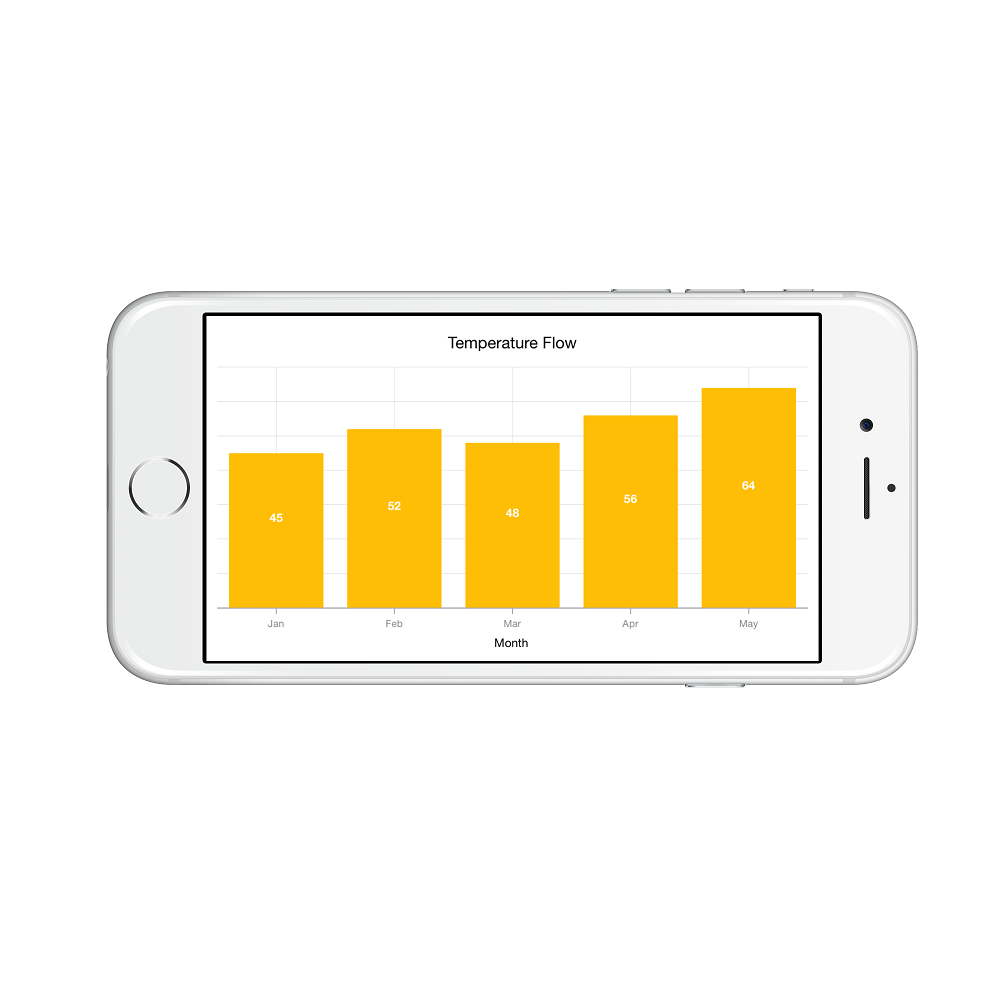### Axis title

The `Title` property in axis provides options to customize the text and font of axis title. Axis does not display title by default. The title can be customized using following properties,

Following code snippet illustrates how to enable and customize the axis title.

• C#
• ``````chart.PrimaryAxis.Title.Text   = new NSString ("Month");

chart.PrimaryAxis.Title.Color  = UIColor.Blue;

chart.PrimaryAxis.Title.Font   = UIFont.BoldSystemFontOfSize(20);``````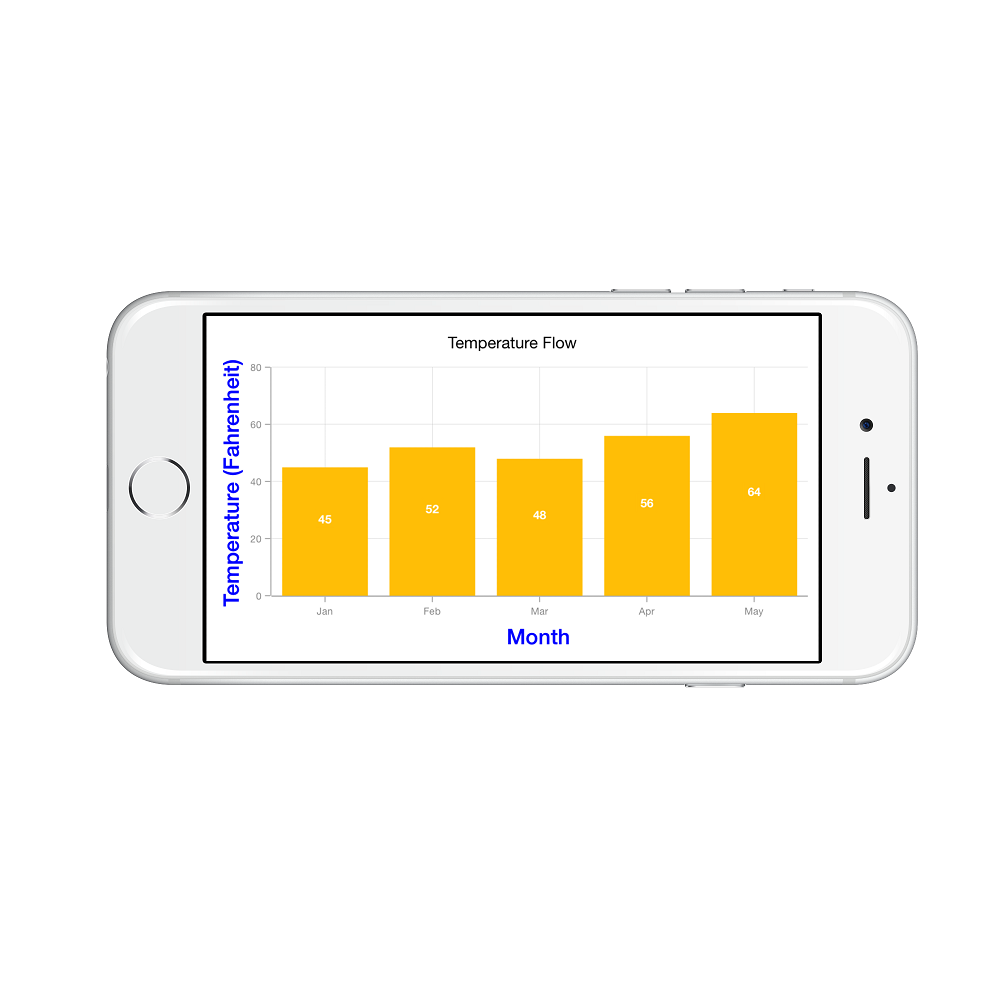### Axis label rotation

The `LabelRotationAngle` property of axis can be used to rotate the axis labels position. Default value of `LabelRotationAngle` property is 0d.

• C#
• ``````SFCategoryAxis categoryAxis = new SFCategoryAxis();

categoryAxis.LabelRotationAngle = -45;

chart.PrimaryAxis = categoryAxis;``````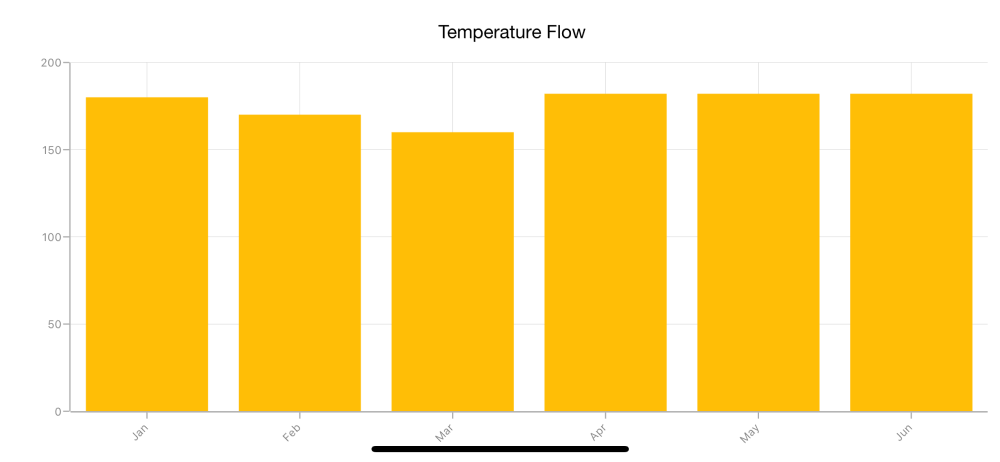### Axis line customization

`SFChart` provides support to customize the style of the axis line by using the `AxisLineStyle` property as shown in the below code snippet.

• C#
• ``````SFCategoryAxis primaryAxis = new SFCategoryAxis();

primaryAxis.AxisLineStyle.LineColor = UIColor.Red;

primaryAxis.AxisLineStyle.LineWidth = 10;

NSObject[] dashes = new NSObject;

dashes  = (NSNumber)2;

dashes  = (NSNumber)3;

primaryAxis.AxisLineStyle.Dashes = NSArray.FromObjects(dashes);

chart.PrimaryAxis = primaryAxis;``````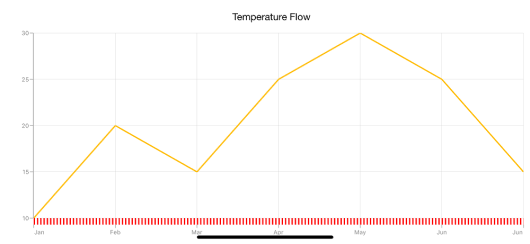### Axis line offset

The `AxisLineOffset` property is used to offset the rendering of axis line.

• C#
• ``````SFCategoryAxis categoryAxis = new SFCategoryAxis();

categoryAxis.PlotOffset = 20;

categoryAxis.AxisLineOffset = 20;

categoryAxis.AxisLineStyle.StrokeWidth = 5;

chart.PrimaryAxis = categoryAxis;``````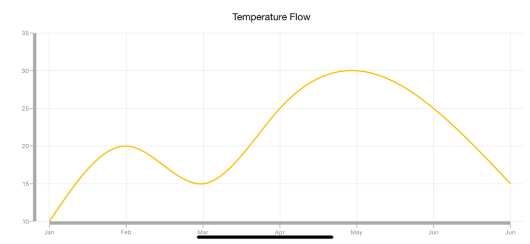### Label customization

The `LabelStyle` property of axis provides options to customize the font-family, color, size and font-weight of axis labels. The axis labels can be customized using following properties:

• C#
• ``````chart.PrimaryAxis.LabelStyle.Font  = UIFont.BoldSystemFontOfSize (20);

chart.PrimaryAxis.LabelStyle.Color = UIColor.Red;``````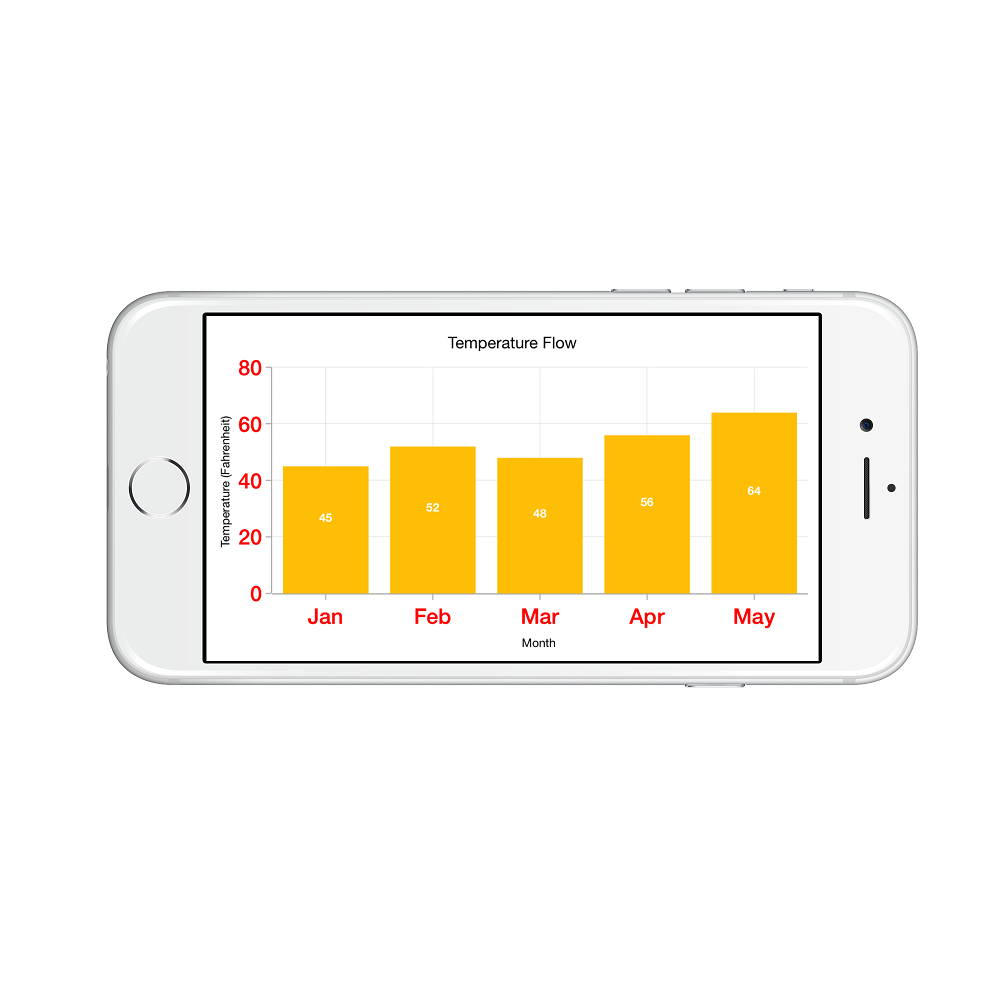### Label and tick positioning

Axis labels and ticks can be positioned `Inside` or `Outside` the chart area by using `LabelsPosition` and `TickPosition` properties of `SFAxis`. By default labels and ticks will be positioned outside the chart area.

• C#
• ``````chart.PrimaryAxis.LabelStyle.LabelsPosition   = SFChartAxisElementPosition.Inside;

chart.PrimaryAxis.TickPosition                = SFChartAxisElementPosition.Inside;``````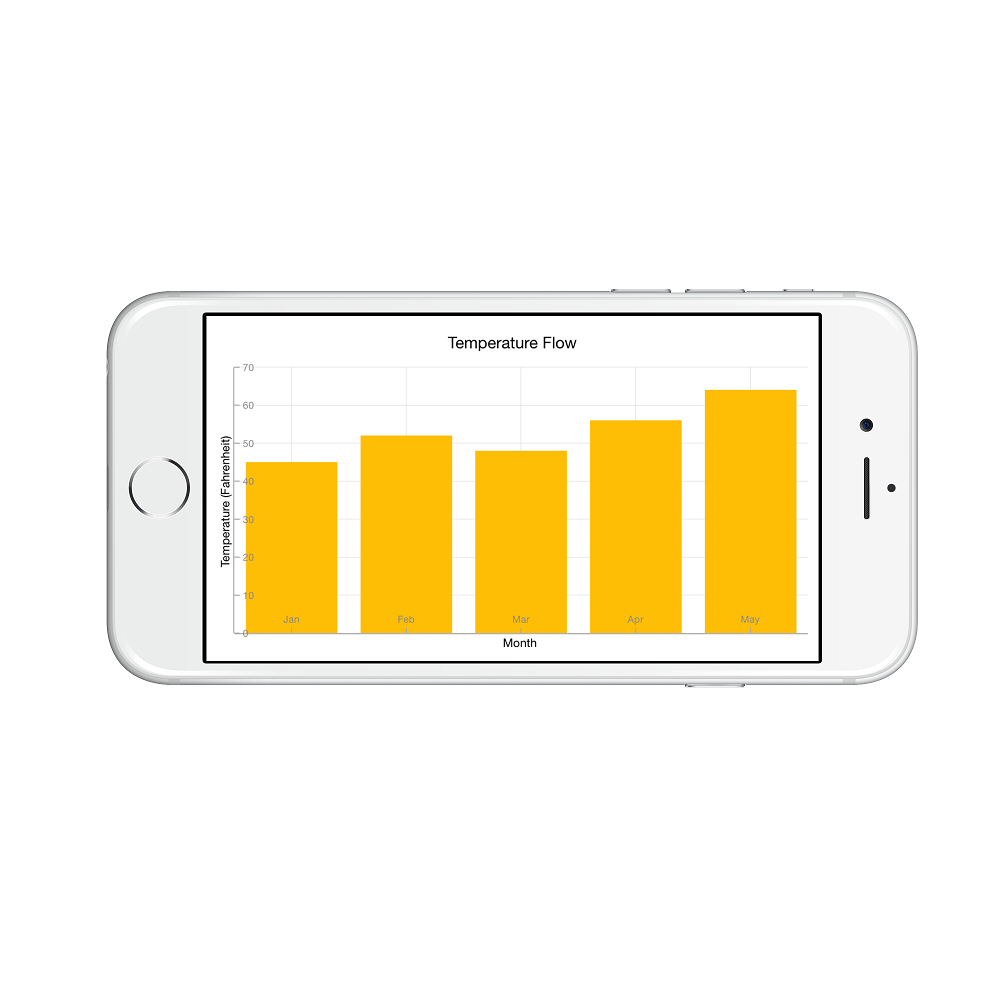### Edge labels placement

Labels with long text at the edges of an axis may appear partially outside the chart. The `EdgeLabelsDrawingMode` property can be used to avoid the partial appearance of labels at the corners. The default `EdgeLabelsDrawingMode` is `Center`.

• C#
• ``chart.PrimaryAxis.EdgeLabelsDrawingMode = SFChartAxisEdgeLabelsDrawingMode.Shift;``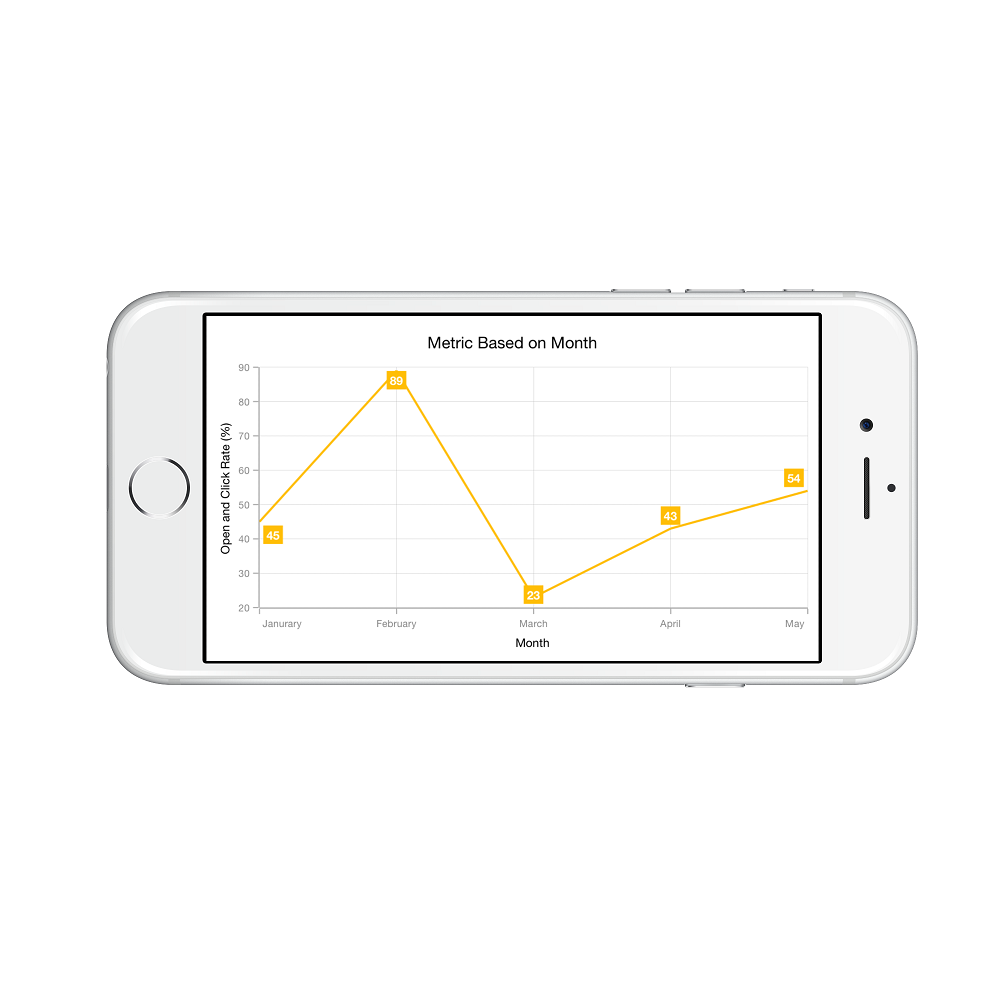### Edge labels visibility

The visibility of the edge labels in an axis can be controlled using `EdgeLabelsVisibilityMode` property.
The following options are available in `EdgeLabelsVisibilityMode`,

• `Default` - used to display the edge label based on auto interval calculations.
• `Visible` - used to display the edge labels (first and last label) irrespective of the auto interval calculation until zooming (i.e., in normal state).
• `AlwaysVisible` - used to always display the edge labels even while zooming the chart.

The following code example demonstrates the AlwaysVisible option.

• C#
• ``chart.SecondaryAxis.EdgeLabelsVisibilityMode  = SFChartAxisEdgeLabelsVisibilityMode.AlwaysVisible;``

### Label extent

The `LabelExtent` property allows to set the gap between axis labels and title. This is typically used to maintain the fixed gap between axis labels and title when the digits of the axis value changed in live update.

• C#
• ``````Chart.PrimaryAxis = new SFCategoryAxis();

Chart.PrimaryAxis.LabelExtent = 60;

Chart.PrimaryAxis.Title.Text = new NSString("Month");``````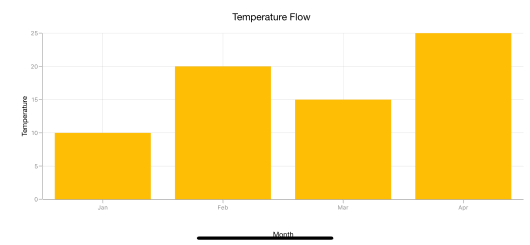### Grid lines customization

The `ShowMajorGridLines` and `ShowMinorGridLines` properties are used to control the visibility of grid lines. `MajorGridLineStyle` and `MinorGridLineStyle` properties in axis are used to customize the major grid lines and minor grid lines of an axis respectively. They provide options to change the width, dashes, color of grid lines. By default minor grid lines will not be visible.

• C#
• ``````yAxis.ShowMajorGridLines    = true;

yAxis.ShowMinorGridLines	= true;

yAxis.MinorTicksPerInterval	= 1;``````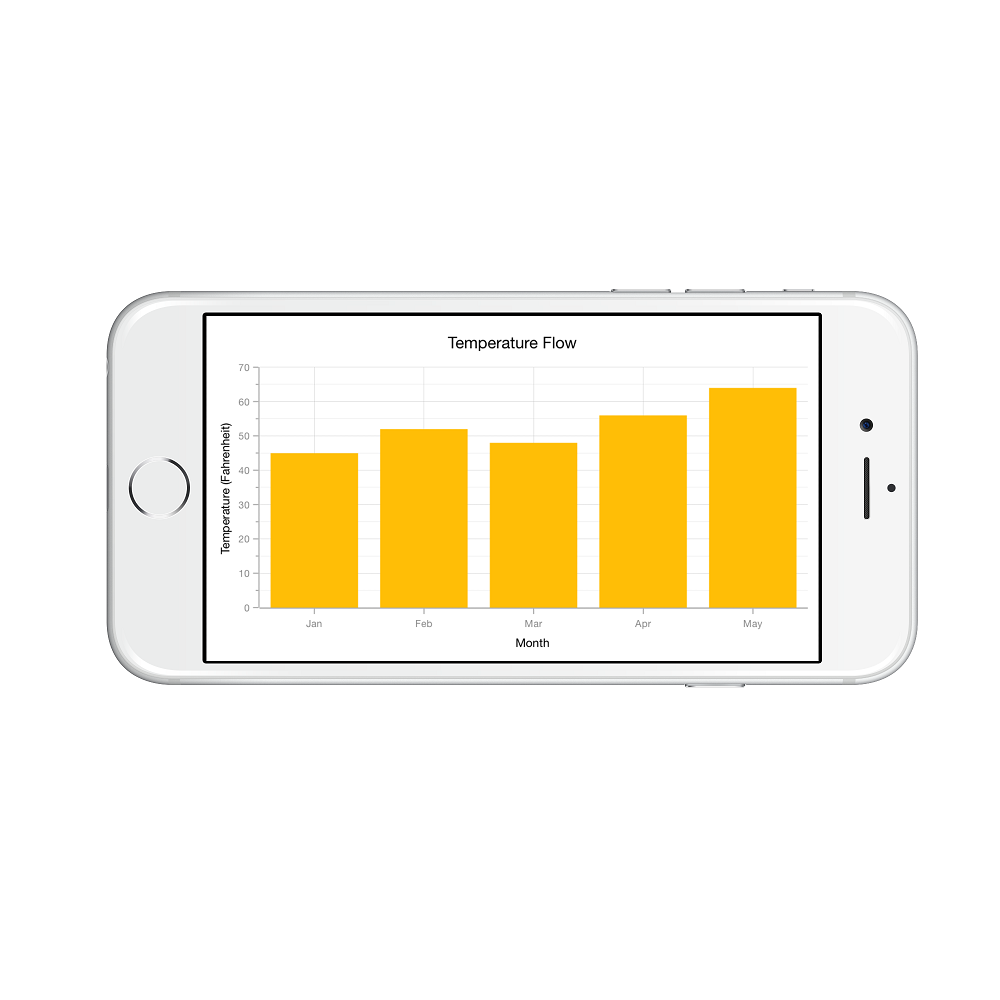### Tick lines customization

The `MajorTickStyle` and `MinorTickStyle` properties in axis are used to customize the major tick lines of an axis and minor tick lines of an axis respectively. They provide options to change the `LineWidth`, `LineSize`, `LineColor` and `MinorTicksPerInterval` of tick lines. By default minor tick lines will not be visible.

• C#
• ``````SFNumericalAxis yAxis           = new SFNumericalAxis ();

chart.SecondaryAxis             = yAxis;

yAxis.MajorTickStyle.LineSize   = new NSNumber (7);

yAxis.MajorTickStyle.LineWidth  = new NSNumber (3);

yAxis.MajorTickStyle.LineColor  = UIColor.Red;

yAxis.ShowMinorGridLines        = true;

yAxis.MinorTicksPerInterval     = 1;

yAxis.MinorTickStyle.LineSize   = new NSNumber (5);

yAxis.MinorTickStyle.LineWidth  = new NSNumber (2);

yAxis.MinorTickStyle.LineColor  = UIColor.Green;``````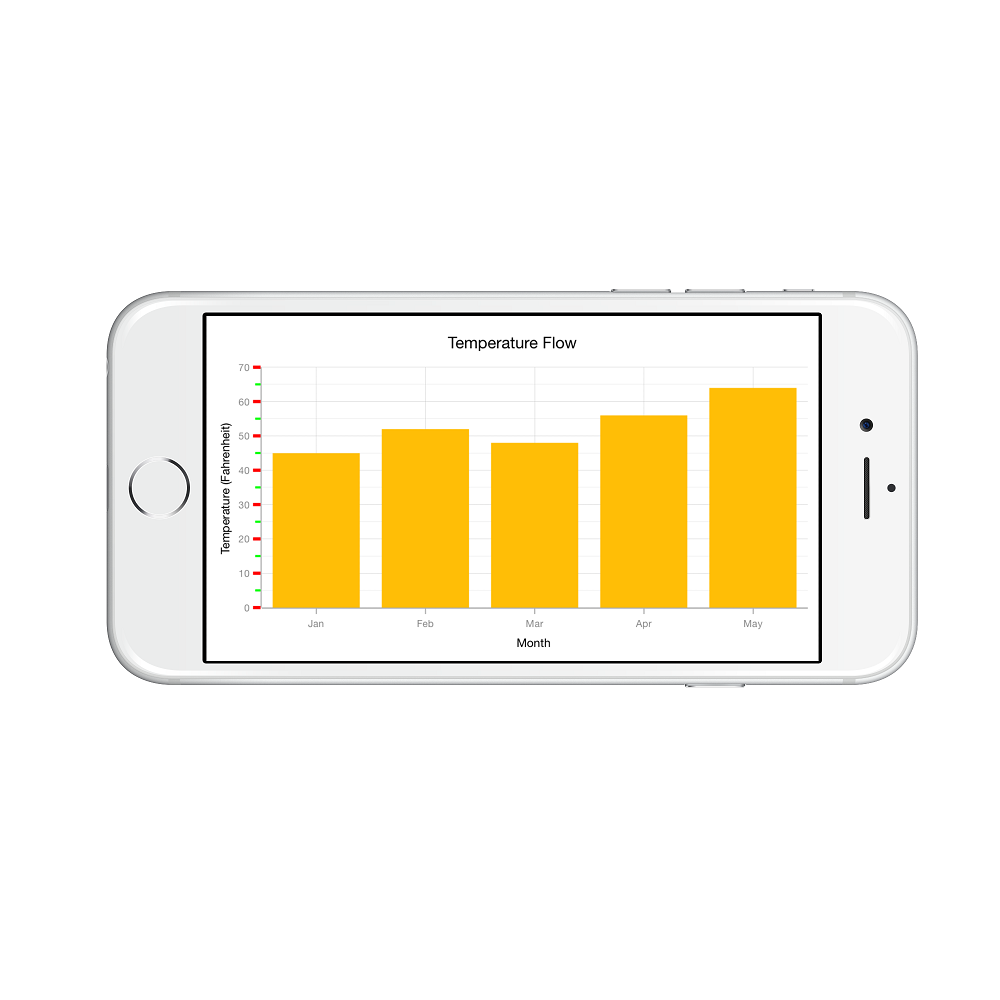### Customize individual axis elements

The `RangeStyles` can be used to customize the gridlines, ticks and axis labels for a specific region of `SFAxis`. The following properties are used to customize the specific range in an axis:

• C#
• ``````SFNumericalAxis  numericalAxis = new SFNumericalAxis () { Minimum = new NSNumber (15), Maximum = new NSNumber (27) };

ChartAxisRangeStyleCollection axisRangeStyles = new ChartAxisRangeStyleCollection();

ChartAxisRangeStyle rangeStyle = new ChartAxisRangeStyle() { Start = 15, End = 21};

rangeStyle.MajorGridLineStyle = new SFAxisGridLineStyle() { LineColor = UIColor.FromRGB(9, 110, 191), LineWidth = 3 };

rangeStyle.LabelStyle = new SFAxisLabelStyle() { Color = UIColor.FromRGB(9, 110, 191)), Font = UIFont.BoldSystemFontOfSize(10) };

....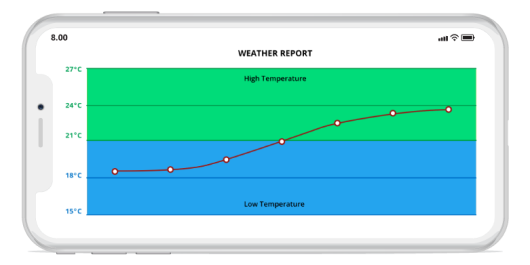### Inversing axis

Axis can be inversed using the `IsInversed` property of axis. Default value of `IsInversed` property is `False`.

• C#
• ``chart.SecondaryAxis.IsInversed = true;``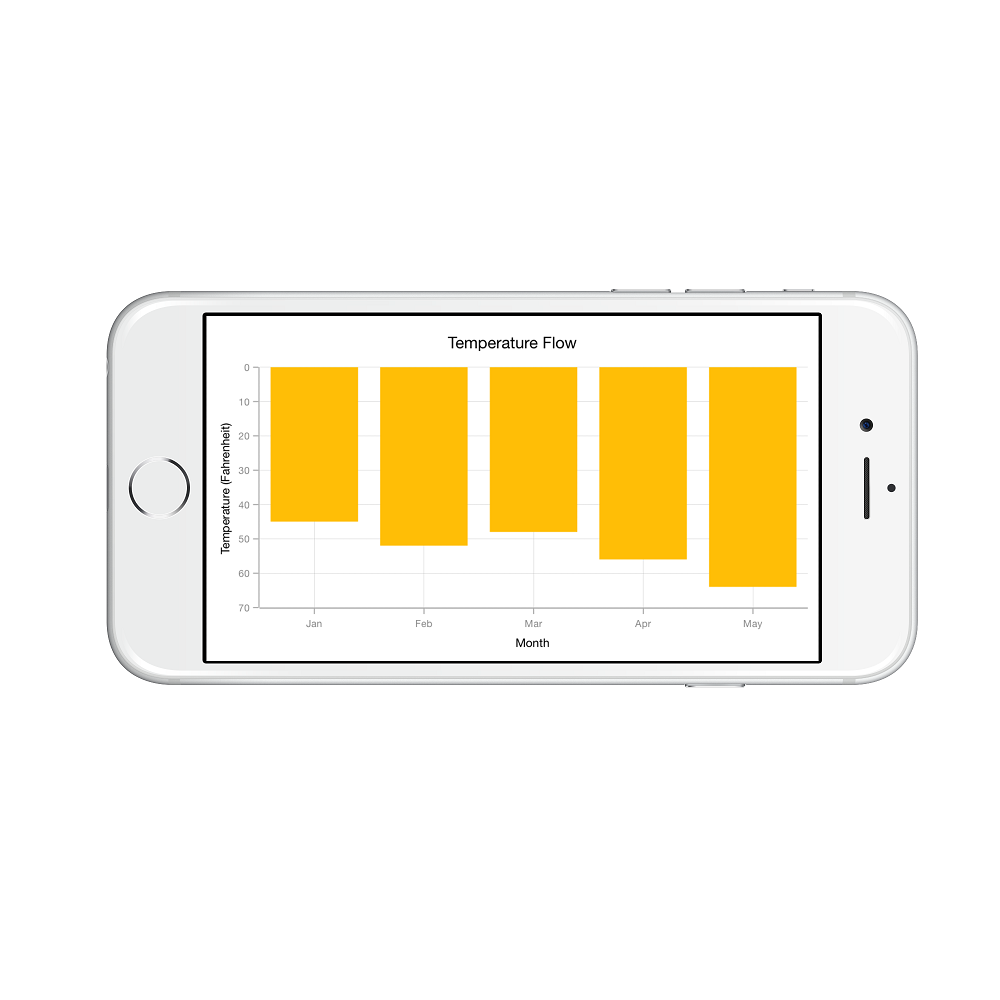### Placing axes at the opposite side

The `OpposedPosition` property of axis can be used to place the axis at the opposite side of its default position. Default value of `OpposedPosition` property is `False`.

• C#
• ``chart.SecondaryAxis.OpposedPosition = true;``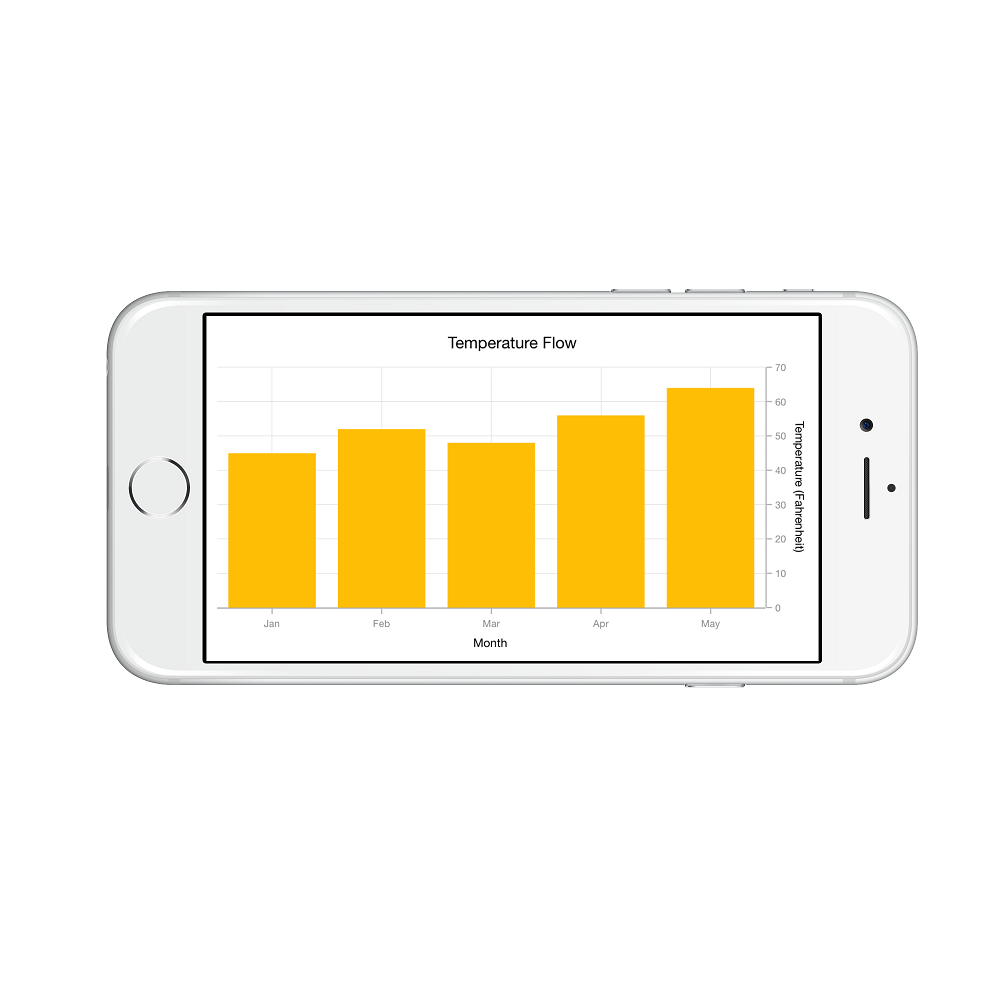### Offset the rendering

The `PlotOffset` property is used to offset the rendering of the axis at start and end position. The following code snippet demonstrates to apply the plot offset to both x and y axes.

• C#
• ``````Chart.PrimaryAxis = new SFCategoryAxis() { PlotOffset = 30 };

Chart.SecondaryAxis = new SFNumericalAxis() { PlotOffset = 30 };``````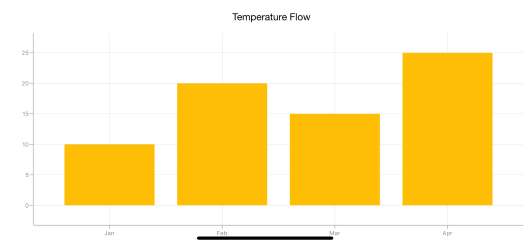#### PlotOffsetStart

The `PlotOffsetStart` property is used to offset the rendering of the axis at start position. The following code snippet demonstrates to apply the plot offset start to both x and y axes.

• C#
• ``````Chart.PrimaryAxis = new SFCategoryAxis() { PlotOffsetStart = 30 };

Chart.SecondaryAxis = new SFNumericalAxis() { PlotOffsetStart = 30 };``````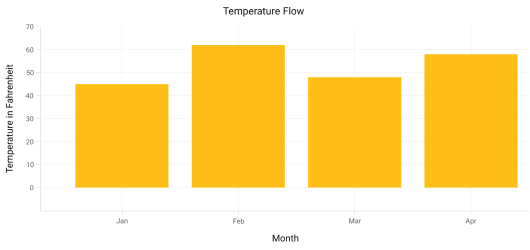#### PlotOffsetEnd

The `PlotOffsetEnd` property is used to offset the rendering of the axis at end position. The following code snippet demonstrates to apply the plot offset end to both x and y axes.

• C#
• ``````Chart.PrimaryAxis = new SFCategoryAxis() { PlotOffsetEnd = 30 };

Chart.SecondaryAxis = new SFNumericalAxis() { PlotOffsetEnd = 30 };``````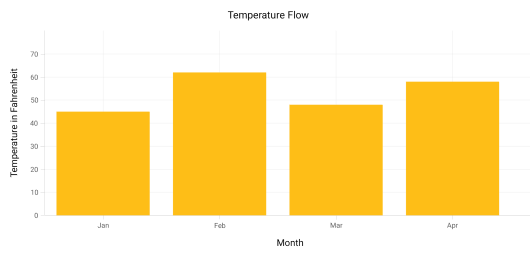### Maximum number of labels per 100 pixels

By default, a maximum of 3 labels are displayed for each 100 pixels in axis. The maximum number of labels that should be present within 100 pixels length can be customized using the `MaximumLabels` property of an axis. This property is applicable only for automatic range calculation and will not work if you set value for `Interval` property of an axis.

• C#
• ``Chart.SecondaryAxis.MaximumLabels = 5;``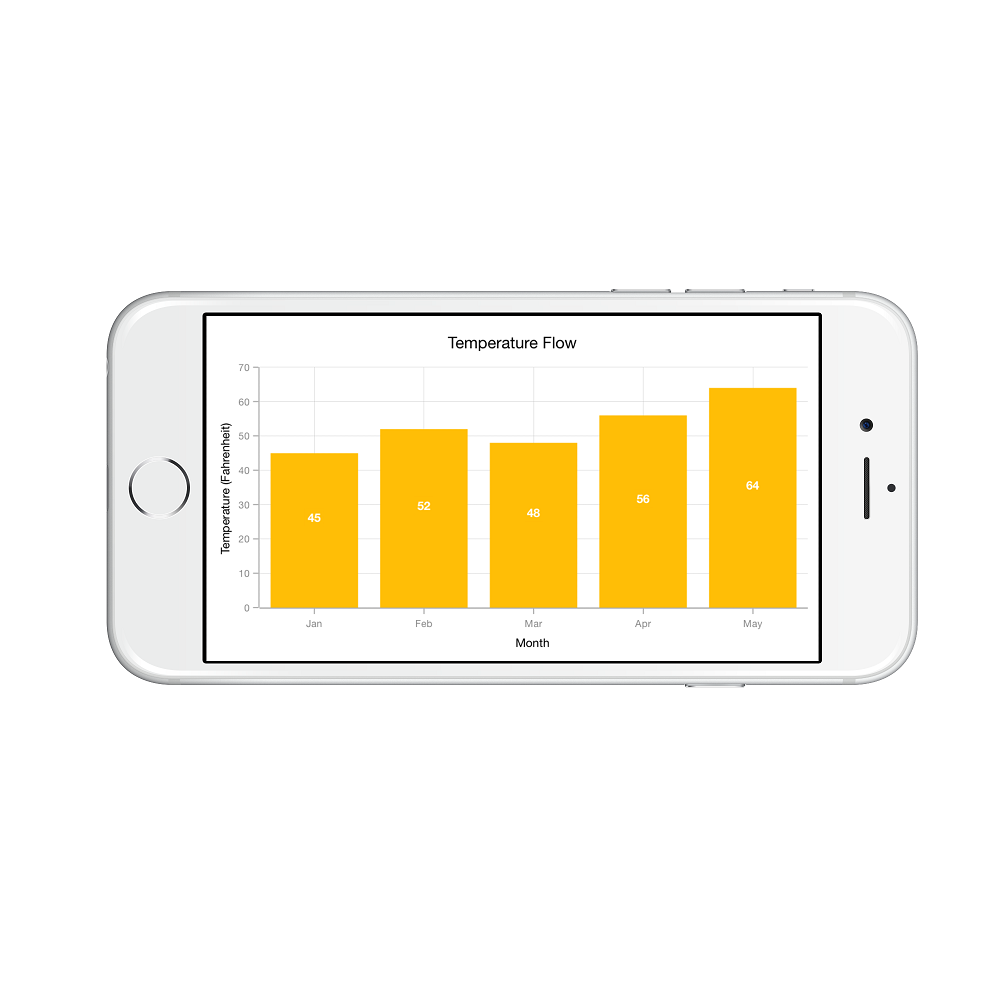### Auto scrolling

`AutoScrollingDelta` property is used to ensure that the specified range of data is always visible in the chart. It always shows the recently added data points at the end and scrolling will be reset to the end of the range whenever a new point is added.

By adding `SFChartZoomPanBehavior` to the chart, you can scroll to see the previous datapoints.

### Auto scrolling delta type

In `SFDateTimeAxis`, you can apply auto scrolling delta value in `Years`, `Months`, `Days`, `Hours`, `Minutes`, `Seconds` and `Milliseconds` by setting `AutoScrollingDeltaType` property.

### Auto scrolling mode

`AutoScrollingMode` property can be used to determine whether the axis should be scrolled from start position or end position. The default value of `AutoScrollingMode` is `End`.

• C#
• ``````SFCategoryAxis primaryAxis = new SFCategoryAxis();

primaryAxis.AutoScrollingDelta = 3;

primaryAxis.AutoScrollingMode = ChartAutoScrollingMode.Start;

chart.PrimaryAxis = primaryAxis;``````

## Axis Crossing

Axis can be positioned anywhere in the chart area by using `CrossesAt` property. This property specifies where the horizontal axis should intersect or cross the vertical axis or vice-versa. Default value of `CrossesAt` property is null.

• C#
• ``````chart.PrimaryAxis  = new SFCategoryAxis ()
{
CrossesAt = 0
};

chart.SecondaryAxis = new SFNumericalAxis()
{
CrossesAt = 8
};``````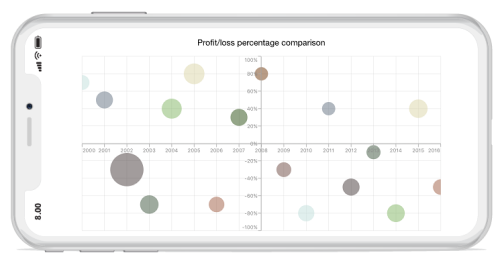### Crossing at specific axis

`CrossingAxisName` property takes axis name as input and determines the axis that used for crossing. By default, all the horizontal axes cross in primary Y axis, and all the vertical axes cross in primary X axis.

• C#
• ``````chart.PrimaryAxis  = new SFCategoryAxis()
{
CrossesAt = 0,

Name = “PrimaryAxis”,

CrossingAxisName = “SecondaryAxis”
};

chart.SecondaryAxis =  new SFNumericalAxis()
{
CrossesAt = 2,

Name = “YAxis”,

CrossingAxisName = “PrimaryAxis”
};

SFBubbleSeries series = new SFBubbleSeries();

series.YAxis = new SFNumericalAxis()
{
CrossesAt = 8,

Name = (NSString)"SecondaryAxis",

CrossingAxisName = (NSString)"PrimaryAxis"
};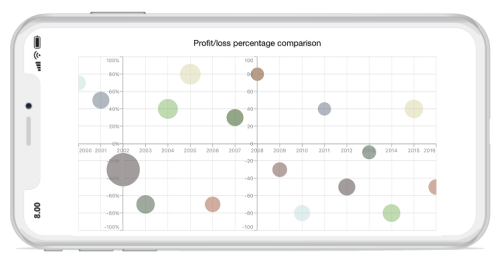### Crossing in date time axis

For crossing in date time horizontal axis, date object should be provided as value for `CrossesAt` property of vertical axis.

• C#
• ``````chart.PrimaryAxis  = new SFDateTimeAxis()
{
CrossesAt = 0
};

chart.SecondaryAxis =  new SFNumericalAxis()
{
CrossesAt = new DateTime(2003, 1, 1)
};``````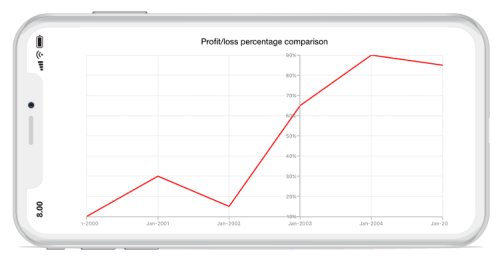### Positioning the axis elements while crossing

The `RenderNextToCrossingValue` property is used to determine whether the crossing axis should be placed at crossing position or not. The default value of `RenderNextToCrossingValue` property is true.

• C#
• ``````chart.PrimaryAxis = new SFCategoryAxis()
{
CrossesAt = 0,

RenderNextToCrossingValue = false

};

chart.SecondaryAxis = new SFNumericalAxis()
{
CrossesAt = 5,
};``````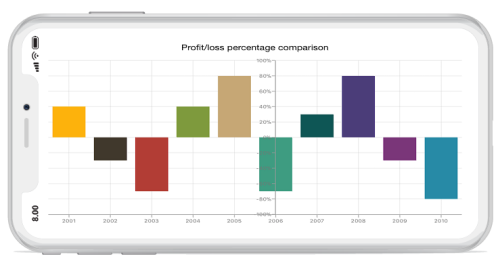## Smart Axis Labels

Axis labels may overlap with each other based on chart dimensions and label size. The `LabelsIntersectAction` property of axis is used to avoid overlapping of axis labels. The default value of the `LabelsIntersectAction` is `SFChartAxisLabelsIntersectAction.None`; other available values are `MultipleRows`, `Hide`, and `Wrap`.

• C#
• ``chart.PrimaryAxis.LabelsIntersectAction = SFChartAxisLabelsIntersectAction.MultipleRows;``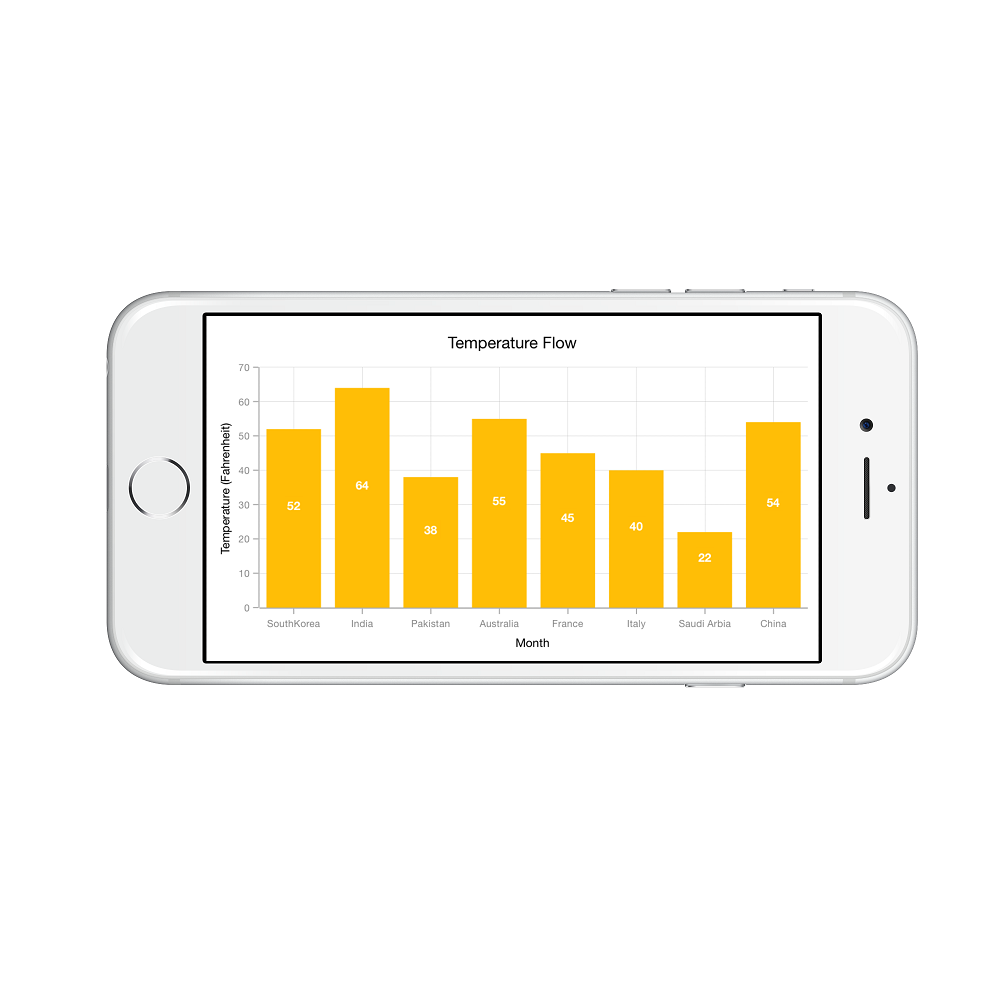## Events

LabelClicked

The `LabelClicked` event is triggered when the axis label is clicked. The argument contains the following information.

• `Label` - Used to get the ChartAxisLabel, which contains axis label position and text.

LabelCreated

The `LabelCreated` event is triggered when the axis label is created. The argument contains `AxisLabel` of `ChartAxisLabel` which contains following properties.